Скачать презентацию Combinatorics Section 6 1 6 6 8 5

ff9aef1eaceb3546c8443a7ed5032535.ppt

• Количество слайдов: 72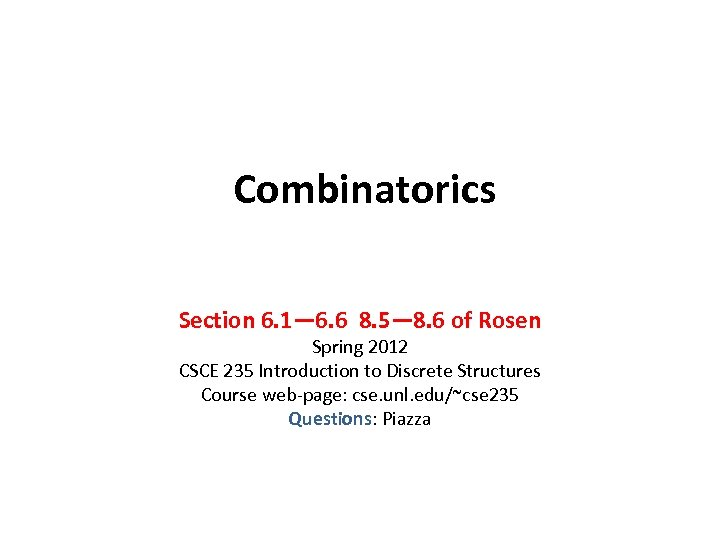Combinatorics Section 6. 1— 6. 6 8. 5— 8. 6 of Rosen Spring 2012 CSCE 235 Introduction to Discrete Structures Course web-page: cse. unl. edu/~cse 235 Questions: Piazza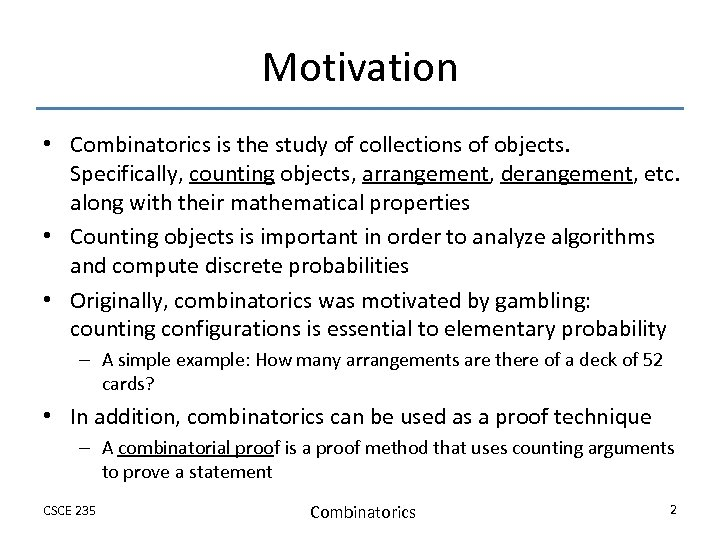Motivation • Combinatorics is the study of collections of objects. Specifically, counting objects, arrangement, derangement, etc. along with their mathematical properties • Counting objects is important in order to analyze algorithms and compute discrete probabilities • Originally, combinatorics was motivated by gambling: counting configurations is essential to elementary probability – A simple example: How many arrangements are there of a deck of 52 cards? • In addition, combinatorics can be used as a proof technique – A combinatorial proof is a proof method that uses counting arguments to prove a statement CSCE 235 Combinatorics 2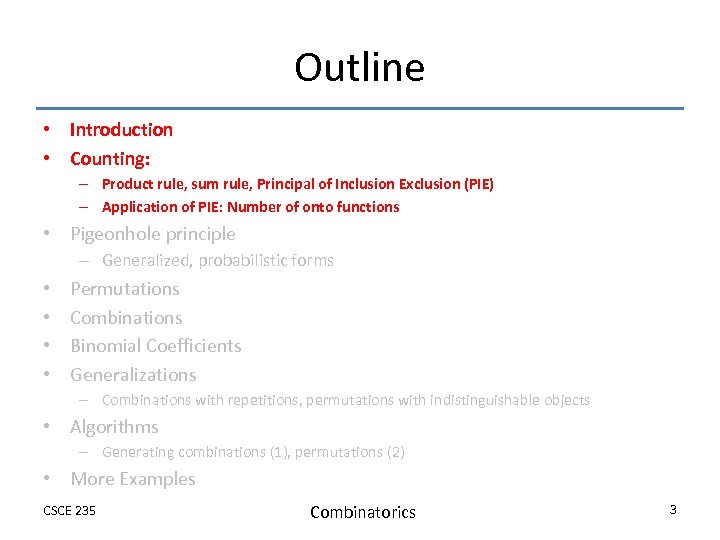Outline • Introduction • Counting: – Product rule, sum rule, Principal of Inclusion Exclusion (PIE) – Application of PIE: Number of onto functions • Pigeonhole principle – Generalized, probabilistic forms • • Permutations Combinations Binomial Coefficients Generalizations – Combinations with repetitions, permutations with indistinguishable objects • Algorithms – Generating combinations (1), permutations (2) • More Examples CSCE 235 Combinatorics 3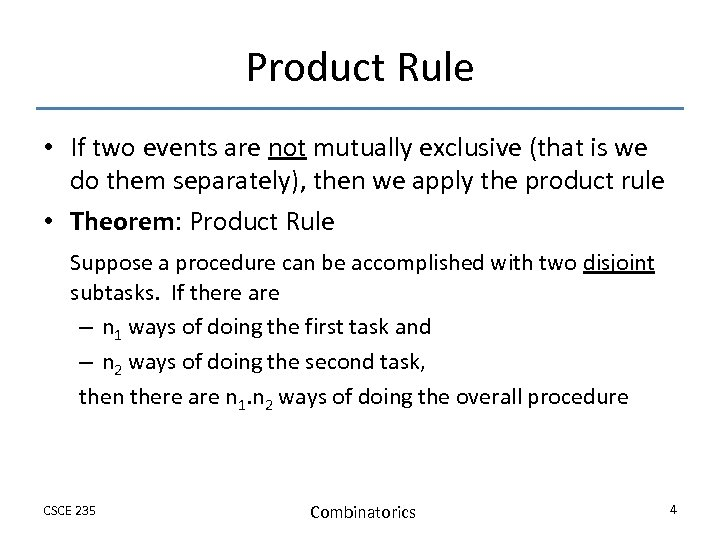Product Rule • If two events are not mutually exclusive (that is we do them separately), then we apply the product rule • Theorem: Product Rule Suppose a procedure can be accomplished with two disjoint subtasks. If there are – n 1 ways of doing the first task and – n 2 ways of doing the second task, then there are n 1. n 2 ways of doing the overall procedure CSCE 235 Combinatorics 4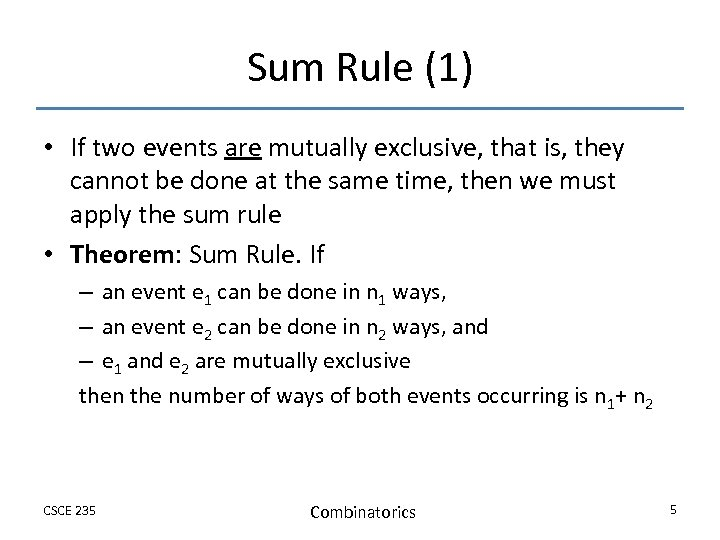Sum Rule (1) • If two events are mutually exclusive, that is, they cannot be done at the same time, then we must apply the sum rule • Theorem: Sum Rule. If – an event e 1 can be done in n 1 ways, – an event e 2 can be done in n 2 ways, and – e 1 and e 2 are mutually exclusive then the number of ways of both events occurring is n 1+ n 2 CSCE 235 Combinatorics 5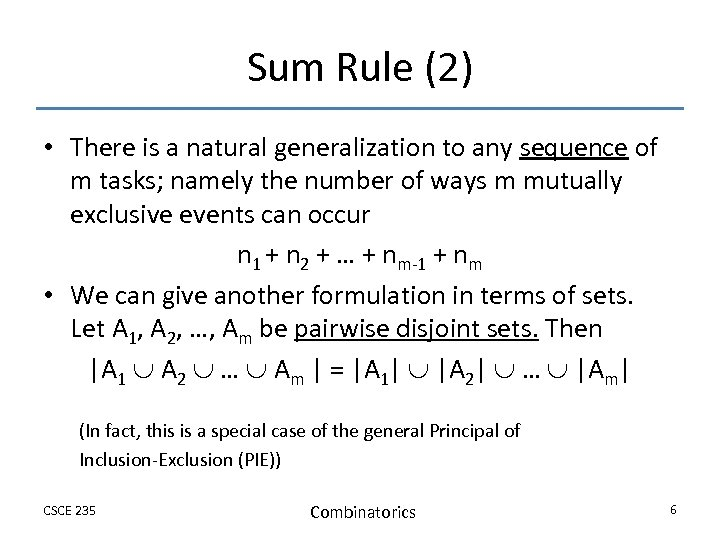Sum Rule (2) • There is a natural generalization to any sequence of m tasks; namely the number of ways m mutually exclusive events can occur n 1 + n 2 + … + nm-1 + nm • We can give another formulation in terms of sets. Let A 1, A 2, …, Am be pairwise disjoint sets. Then |A 1 A 2 … Am | = |A 1| |A 2| … |Am| (In fact, this is a special case of the general Principal of Inclusion-Exclusion (PIE)) CSCE 235 Combinatorics 6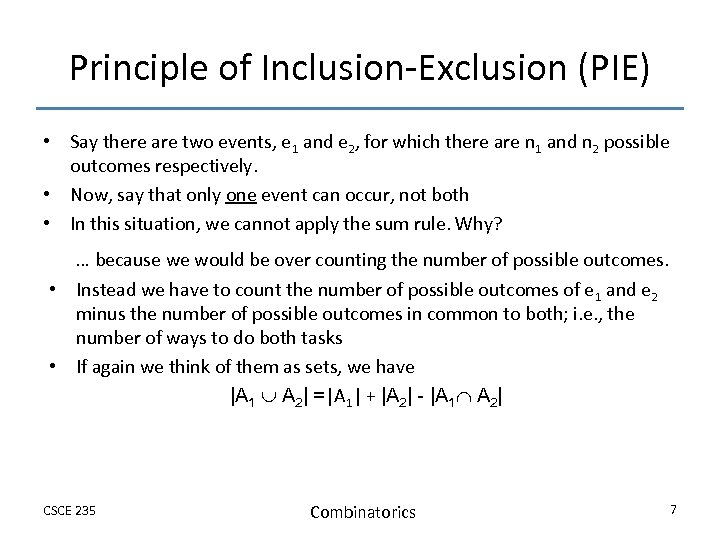Principle of Inclusion-Exclusion (PIE) • Say there are two events, e 1 and e 2, for which there are n 1 and n 2 possible outcomes respectively. • Now, say that only one event can occur, not both • In this situation, we cannot apply the sum rule. Why? … because we would be over counting the number of possible outcomes. • Instead we have to count the number of possible outcomes of e 1 and e 2 minus the number of possible outcomes in common to both; i. e. , the number of ways to do both tasks • If again we think of them as sets, we have |A 1 A 2| =|A 1| + |A 2| - |A 1 A 2| CSCE 235 Combinatorics 7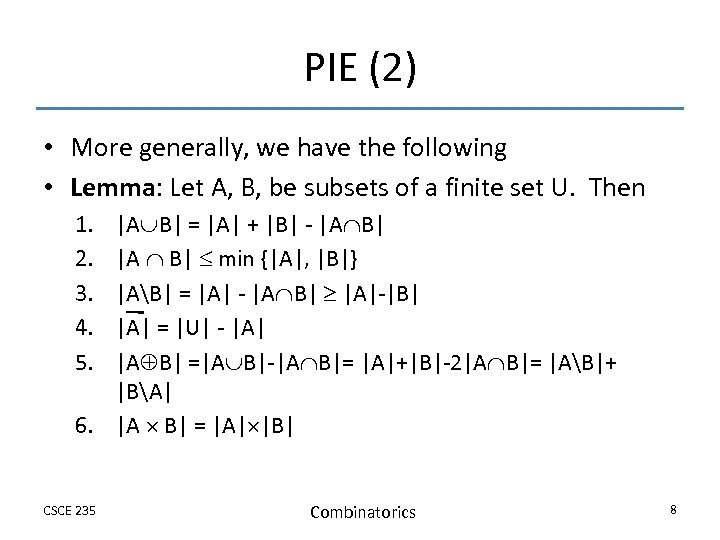PIE (2) • More generally, we have the following • Lemma: Let A, B, be subsets of a finite set U. Then 1. 2. 3. 4. 5. |A B| = |A| + |B| - |A B| |A B| min {|A|, |B|} |AB| = |A| - |A B| |A|-|B| |A| = |U| - |A| |A B| =|A B|-|A B|= |A|+|B|-2|A B|= |AB|+ |BA| 6. |A B| = |A| |B| CSCE 235 Combinatorics 8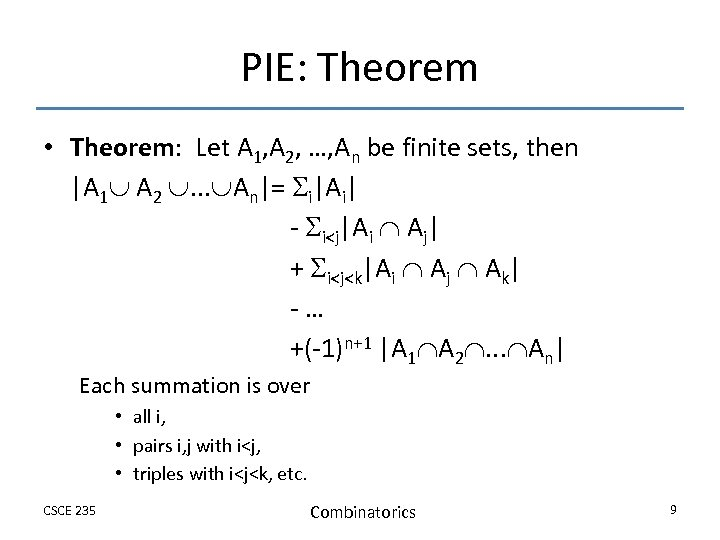PIE: Theorem • Theorem: Let A 1, A 2, …, An be finite sets, then |A 1 A 2 . . . An|= i|Ai| - i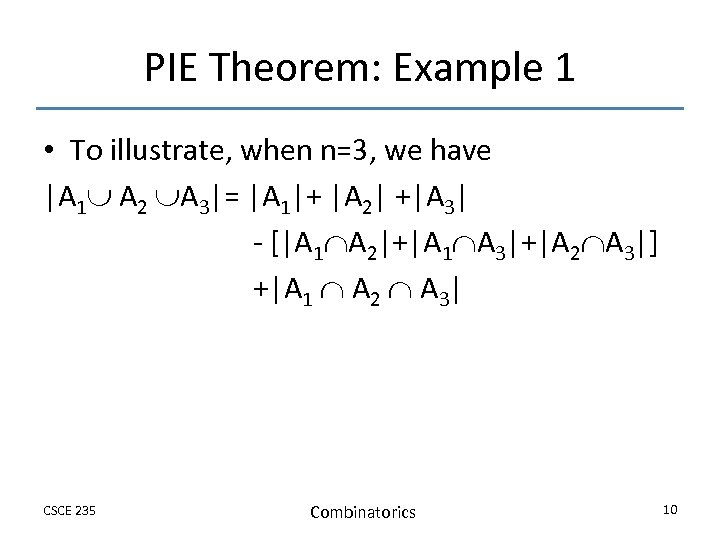PIE Theorem: Example 1 • To illustrate, when n=3, we have |A 1 A 2 A 3|= |A 1|+ |A 2| +|A 3| - [|A 1 A 2|+|A 1 A 3|+|A 2 A 3|] +|A 1 A 2 A 3| CSCE 235 Combinatorics 10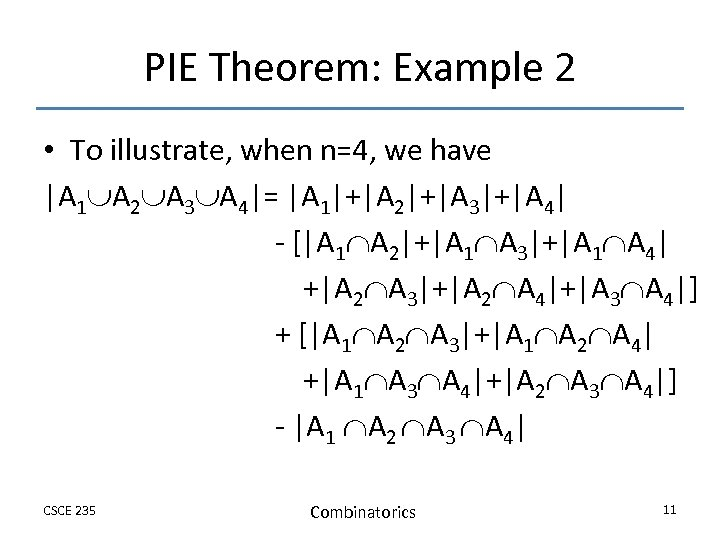PIE Theorem: Example 2 • To illustrate, when n=4, we have |A 1 A 2 A 3 A 4|= |A 1|+|A 2|+|A 3|+|A 4| - [|A 1 A 2|+|A 1 A 3|+|A 1 A 4| +|A 2 A 3|+|A 2 A 4|+|A 3 A 4|] + [|A 1 A 2 A 3|+|A 1 A 2 A 4| +|A 1 A 3 A 4|+|A 2 A 3 A 4|] - |A 1 A 2 A 3 A 4| CSCE 235 Combinatorics 11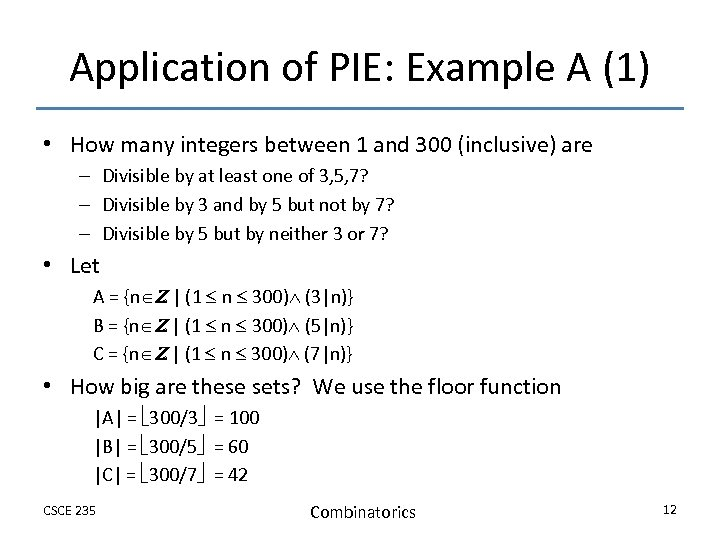Application of PIE: Example A (1) • How many integers between 1 and 300 (inclusive) are – Divisible by at least one of 3, 5, 7? – Divisible by 3 and by 5 but not by 7? – Divisible by 5 but by neither 3 or 7? • Let A = {n Z | (1 n 300) (3|n)} B = {n Z | (1 n 300) (5|n)} C = {n Z | (1 n 300) (7|n)} • How big are these sets? We use the floor function |A| = 300/3 = 100 |B| = 300/5 = 60 |C| = 300/7 = 42 CSCE 235 Combinatorics 12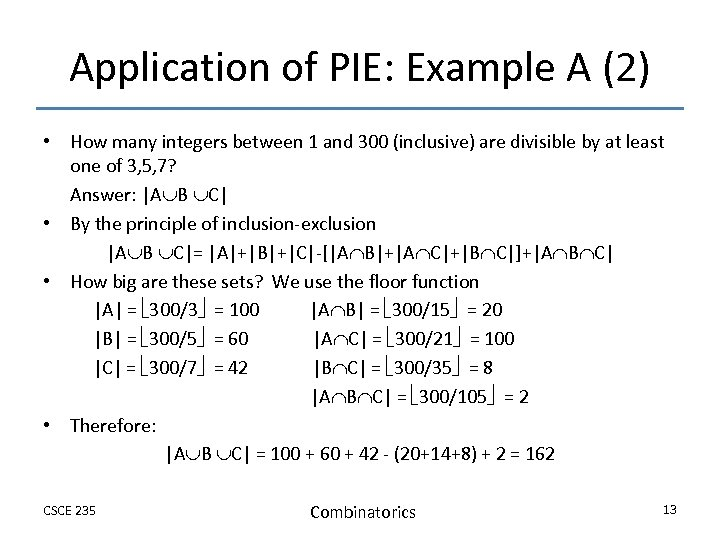Application of PIE: Example A (2) • How many integers between 1 and 300 (inclusive) are divisible by at least one of 3, 5, 7? Answer: |A B C| • By the principle of inclusion-exclusion |A B C|= |A|+|B|+|C|-[|A B|+|A C|+|B C|]+|A B C| • How big are these sets? We use the floor function |A| = 300/3 = 100 |A B| = 300/15 = 20 |B| = 300/5 = 60 |A C| = 300/21 = 100 |C| = 300/7 = 42 |B C| = 300/35 = 8 |A B C| = 300/105 = 2 • Therefore: |A B C| = 100 + 60 + 42 - (20+14+8) + 2 = 162 CSCE 235 Combinatorics 13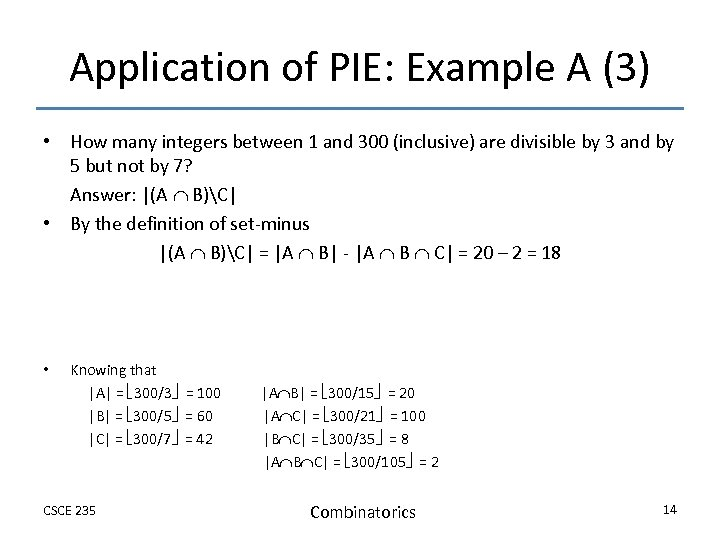Application of PIE: Example A (3) • How many integers between 1 and 300 (inclusive) are divisible by 3 and by 5 but not by 7? Answer: |(A B)C| • By the definition of set-minus |(A B)C| = |A B| - |A B C| = 20 – 2 = 18 • Knowing that |A| = 300/3 = 100 |B| = 300/5 = 60 |C| = 300/7 = 42 CSCE 235 |A B| = 300/15 = 20 |A C| = 300/21 = 100 |B C| = 300/35 = 8 |A B C| = 300/105 = 2 Combinatorics 14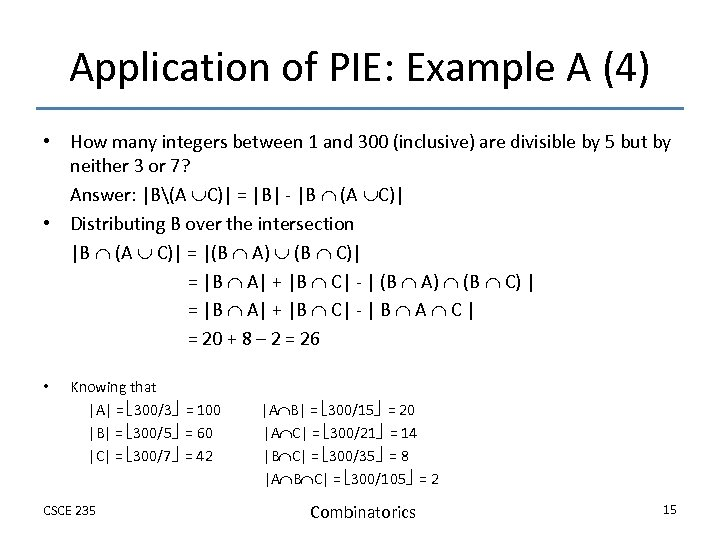Application of PIE: Example A (4) • How many integers between 1 and 300 (inclusive) are divisible by 5 but by neither 3 or 7? Answer: |B(A C)| = |B| - |B (A C)| • Distributing B over the intersection |B (A C)| = |(B A) (B C)| = |B A| + |B C| - | (B A) (B C) | = |B A| + |B C| - | B A C | = 20 + 8 – 2 = 26 • Knowing that |A| = 300/3 = 100 |B| = 300/5 = 60 |C| = 300/7 = 42 CSCE 235 |A B| = 300/15 = 20 |A C| = 300/21 = 14 |B C| = 300/35 = 8 |A B C| = 300/105 = 2 Combinatorics 15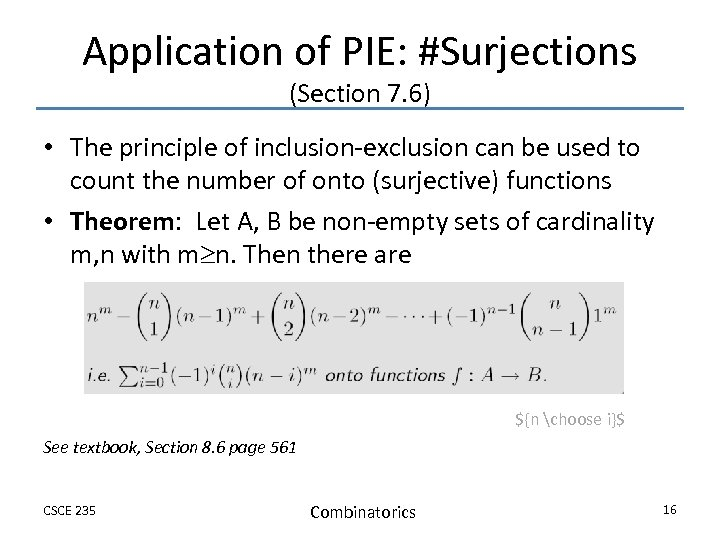Application of PIE: #Surjections (Section 7. 6) • The principle of inclusion-exclusion can be used to count the number of onto (surjective) functions • Theorem: Let A, B be non-empty sets of cardinality m, n with m n. Then there are \${n choose i}\$ See textbook, Section 8. 6 page 561 CSCE 235 Combinatorics 16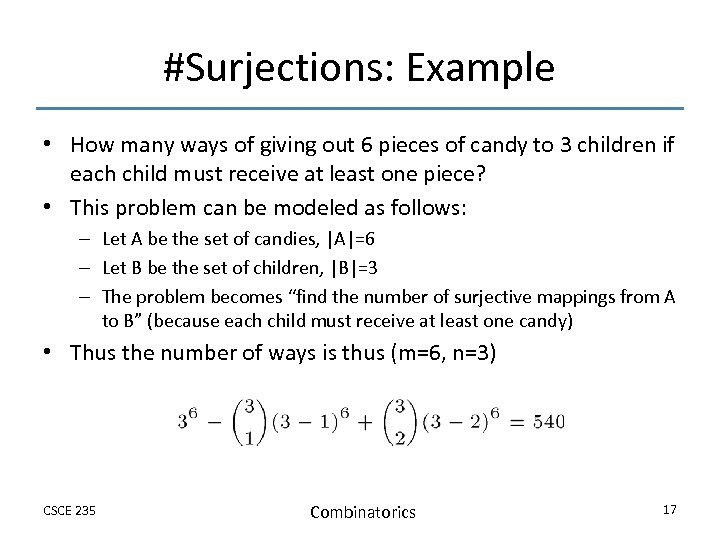#Surjections: Example • How many ways of giving out 6 pieces of candy to 3 children if each child must receive at least one piece? • This problem can be modeled as follows: – Let A be the set of candies, |A|=6 – Let B be the set of children, |B|=3 – The problem becomes “find the number of surjective mappings from A to B” (because each child must receive at least one candy) • Thus the number of ways is thus (m=6, n=3) CSCE 235 Combinatorics 17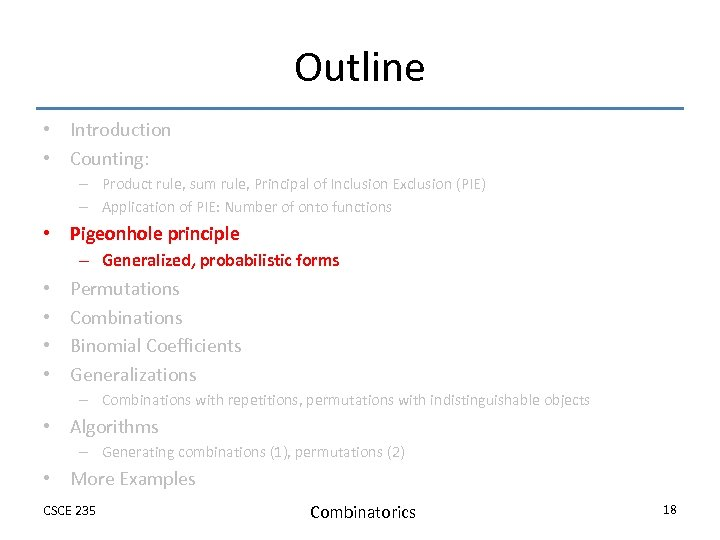Outline • Introduction • Counting: – Product rule, sum rule, Principal of Inclusion Exclusion (PIE) – Application of PIE: Number of onto functions • Pigeonhole principle – Generalized, probabilistic forms • • Permutations Combinations Binomial Coefficients Generalizations – Combinations with repetitions, permutations with indistinguishable objects • Algorithms – Generating combinations (1), permutations (2) • More Examples CSCE 235 Combinatorics 18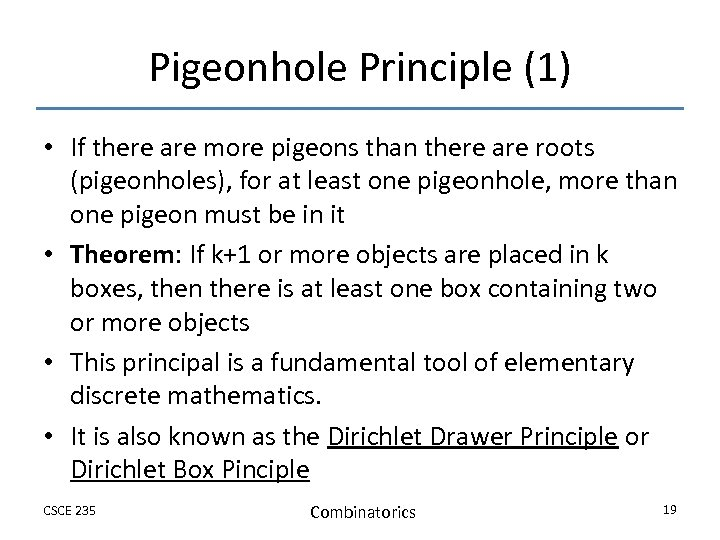Pigeonhole Principle (1) • If there are more pigeons than there are roots (pigeonholes), for at least one pigeonhole, more than one pigeon must be in it • Theorem: If k+1 or more objects are placed in k boxes, then there is at least one box containing two or more objects • This principal is a fundamental tool of elementary discrete mathematics. • It is also known as the Dirichlet Drawer Principle or Dirichlet Box Pinciple CSCE 235 Combinatorics 19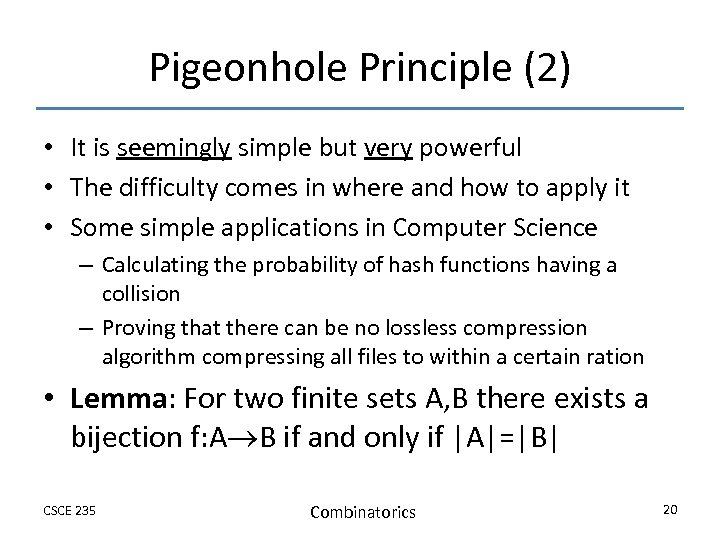Pigeonhole Principle (2) • It is seemingly simple but very powerful • The difficulty comes in where and how to apply it • Some simple applications in Computer Science – Calculating the probability of hash functions having a collision – Proving that there can be no lossless compression algorithm compressing all files to within a certain ration • Lemma: For two finite sets A, B there exists a bijection f: A B if and only if |A|=|B| CSCE 235 Combinatorics 20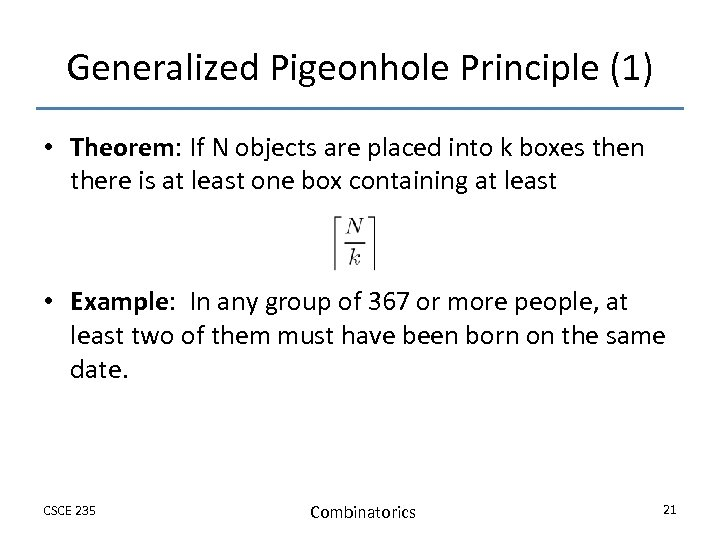Generalized Pigeonhole Principle (1) • Theorem: If N objects are placed into k boxes then there is at least one box containing at least • Example: In any group of 367 or more people, at least two of them must have been born on the same date. CSCE 235 Combinatorics 21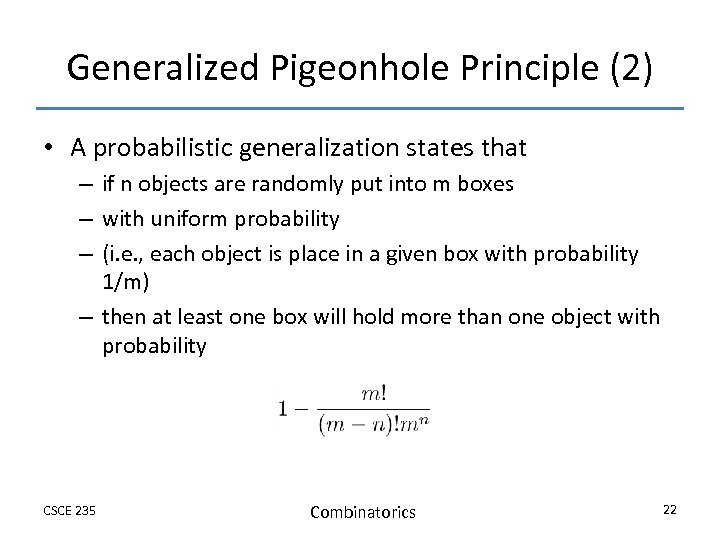Generalized Pigeonhole Principle (2) • A probabilistic generalization states that – if n objects are randomly put into m boxes – with uniform probability – (i. e. , each object is place in a given box with probability 1/m) – then at least one box will hold more than one object with probability CSCE 235 Combinatorics 22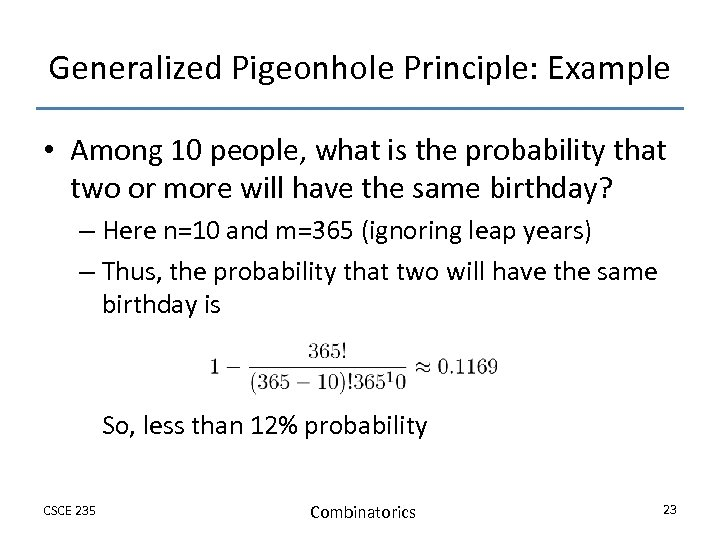Generalized Pigeonhole Principle: Example • Among 10 people, what is the probability that two or more will have the same birthday? – Here n=10 and m=365 (ignoring leap years) – Thus, the probability that two will have the same birthday is So, less than 12% probability CSCE 235 Combinatorics 23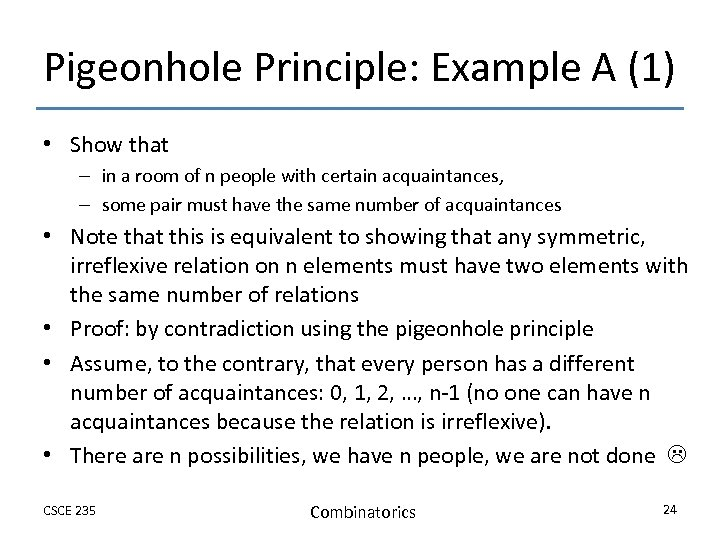Pigeonhole Principle: Example A (1) • Show that – in a room of n people with certain acquaintances, – some pair must have the same number of acquaintances • Note that this is equivalent to showing that any symmetric, irreflexive relation on n elements must have two elements with the same number of relations • Proof: by contradiction using the pigeonhole principle • Assume, to the contrary, that every person has a different number of acquaintances: 0, 1, 2, …, n-1 (no one can have n acquaintances because the relation is irreflexive). • There are n possibilities, we have n people, we are not done CSCE 235 Combinatorics 24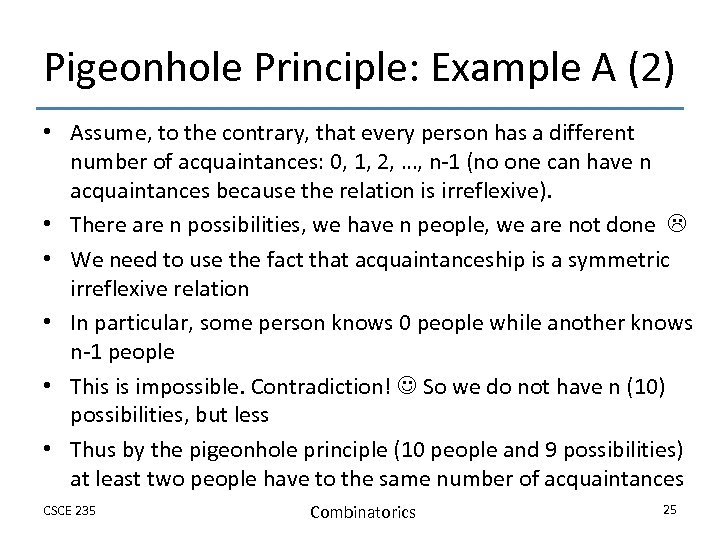Pigeonhole Principle: Example A (2) • Assume, to the contrary, that every person has a different number of acquaintances: 0, 1, 2, …, n-1 (no one can have n acquaintances because the relation is irreflexive). • There are n possibilities, we have n people, we are not done • We need to use the fact that acquaintanceship is a symmetric irreflexive relation • In particular, some person knows 0 people while another knows n-1 people • This is impossible. Contradiction! So we do not have n (10) possibilities, but less • Thus by the pigeonhole principle (10 people and 9 possibilities) at least two people have to the same number of acquaintances CSCE 235 Combinatorics 25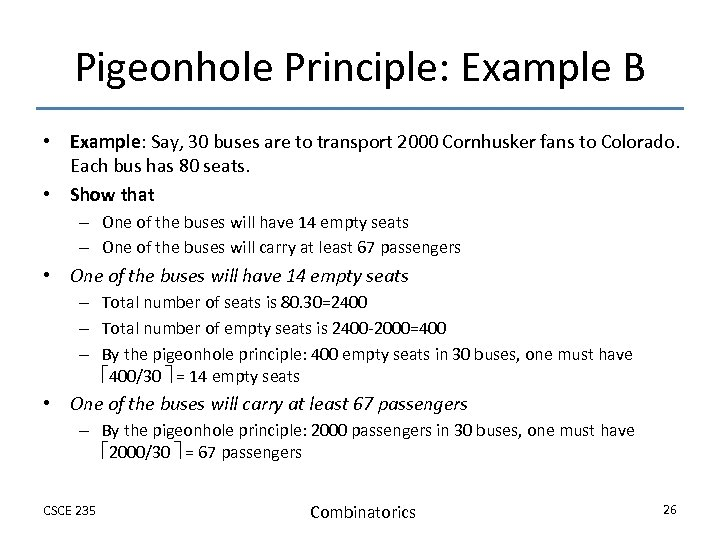Pigeonhole Principle: Example B • Example: Say, 30 buses are to transport 2000 Cornhusker fans to Colorado. Each bus has 80 seats. • Show that – One of the buses will have 14 empty seats – One of the buses will carry at least 67 passengers • One of the buses will have 14 empty seats – Total number of seats is 80. 30=2400 – Total number of empty seats is 2400 -2000=400 – By the pigeonhole principle: 400 empty seats in 30 buses, one must have 400/30 = 14 empty seats • One of the buses will carry at least 67 passengers – By the pigeonhole principle: 2000 passengers in 30 buses, one must have 2000/30 = 67 passengers CSCE 235 Combinatorics 26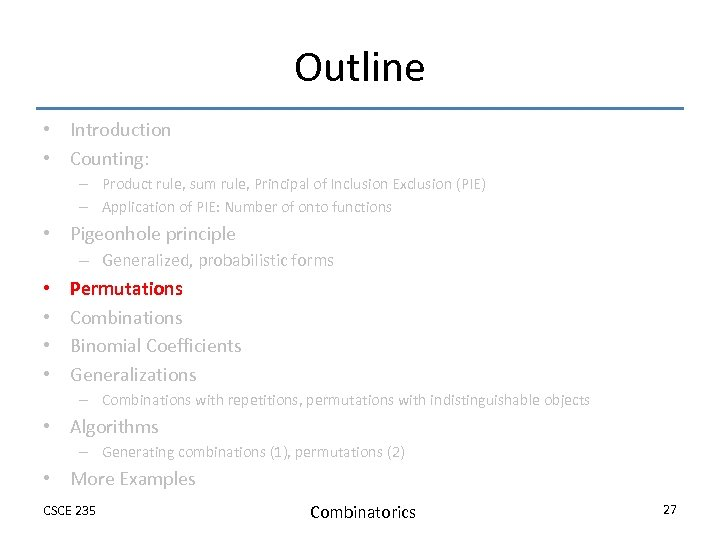Outline • Introduction • Counting: – Product rule, sum rule, Principal of Inclusion Exclusion (PIE) – Application of PIE: Number of onto functions • Pigeonhole principle – Generalized, probabilistic forms • • Permutations Combinations Binomial Coefficients Generalizations – Combinations with repetitions, permutations with indistinguishable objects • Algorithms – Generating combinations (1), permutations (2) • More Examples CSCE 235 Combinatorics 27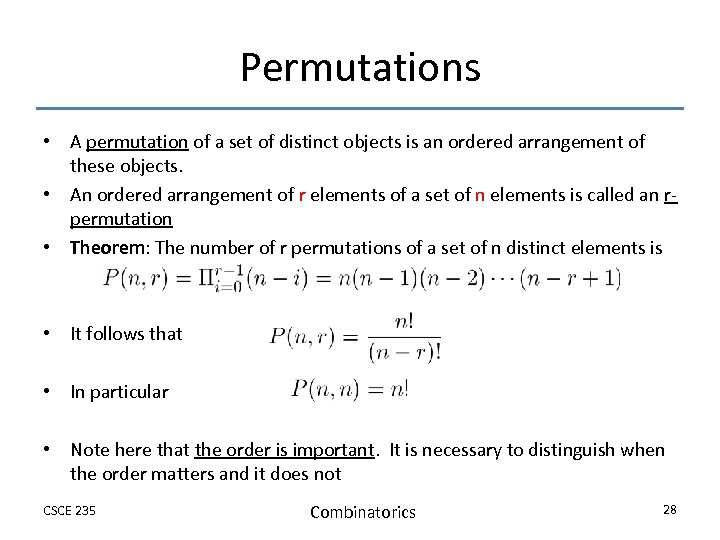Permutations • A permutation of a set of distinct objects is an ordered arrangement of these objects. • An ordered arrangement of r elements of a set of n elements is called an rpermutation • Theorem: The number of r permutations of a set of n distinct elements is • It follows that • In particular • Note here that the order is important. It is necessary to distinguish when the order matters and it does not CSCE 235 Combinatorics 28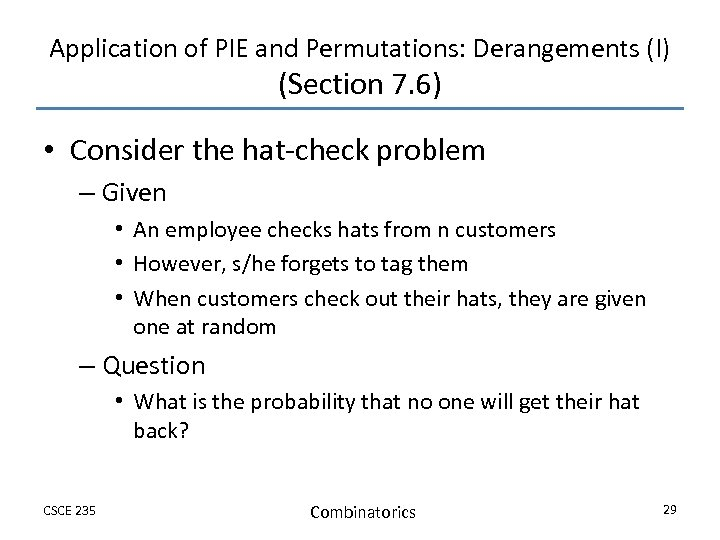Application of PIE and Permutations: Derangements (I) (Section 7. 6) • Consider the hat-check problem – Given • An employee checks hats from n customers • However, s/he forgets to tag them • When customers check out their hats, they are given one at random – Question • What is the probability that no one will get their hat back? CSCE 235 Combinatorics 29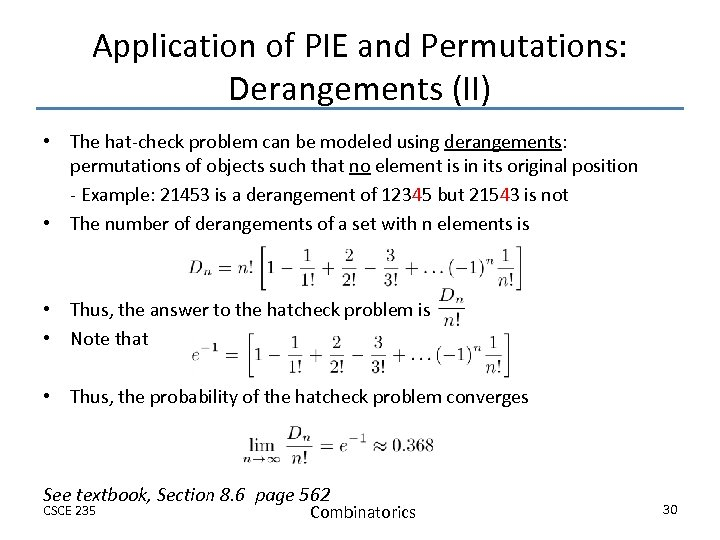Application of PIE and Permutations: Derangements (II) • The hat-check problem can be modeled using derangements: permutations of objects such that no element is in its original position - Example: 21453 is a derangement of 12345 but 21543 is not • The number of derangements of a set with n elements is • Thus, the answer to the hatcheck problem is • Note that • Thus, the probability of the hatcheck problem converges See textbook, Section 8. 6 page 562 CSCE 235 Combinatorics 30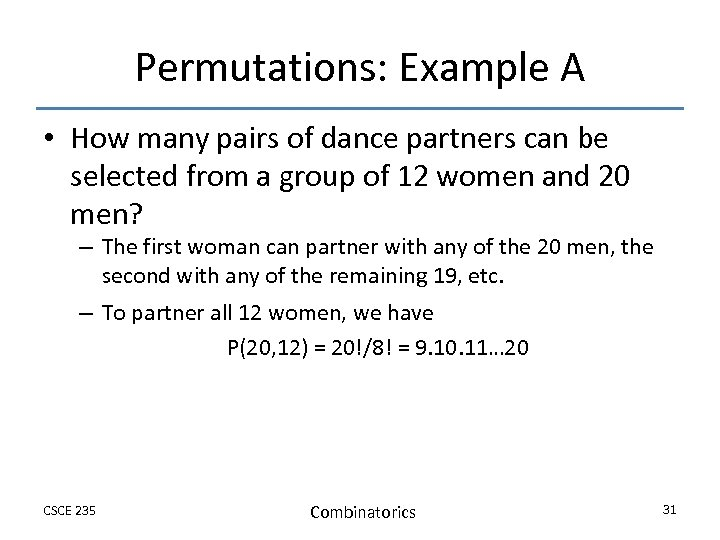Permutations: Example A • How many pairs of dance partners can be selected from a group of 12 women and 20 men? – The first woman can partner with any of the 20 men, the second with any of the remaining 19, etc. – To partner all 12 women, we have P(20, 12) = 20!/8! = 9. 10. 11… 20 CSCE 235 Combinatorics 31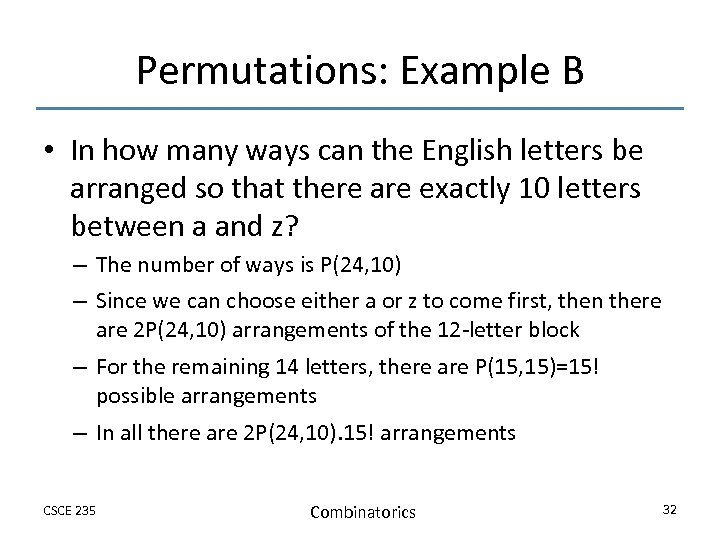Permutations: Example B • In how many ways can the English letters be arranged so that there are exactly 10 letters between a and z? – The number of ways is P(24, 10) – Since we can choose either a or z to come first, then there are 2 P(24, 10) arrangements of the 12 -letter block – For the remaining 14 letters, there are P(15, 15)=15! possible arrangements – In all there are 2 P(24, 10). 15! arrangements CSCE 235 Combinatorics 32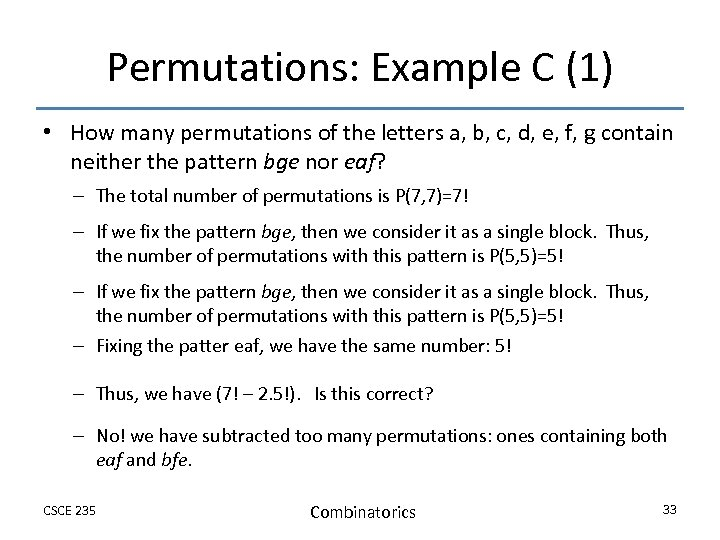Permutations: Example C (1) • How many permutations of the letters a, b, c, d, e, f, g contain neither the pattern bge nor eaf? – The total number of permutations is P(7, 7)=7! – If we fix the pattern bge, then we consider it as a single block. Thus, the number of permutations with this pattern is P(5, 5)=5! – Fixing the patter eaf, we have the same number: 5! – Thus, we have (7! – 2. 5!). Is this correct? – No! we have subtracted too many permutations: ones containing both eaf and bfe. CSCE 235 Combinatorics 33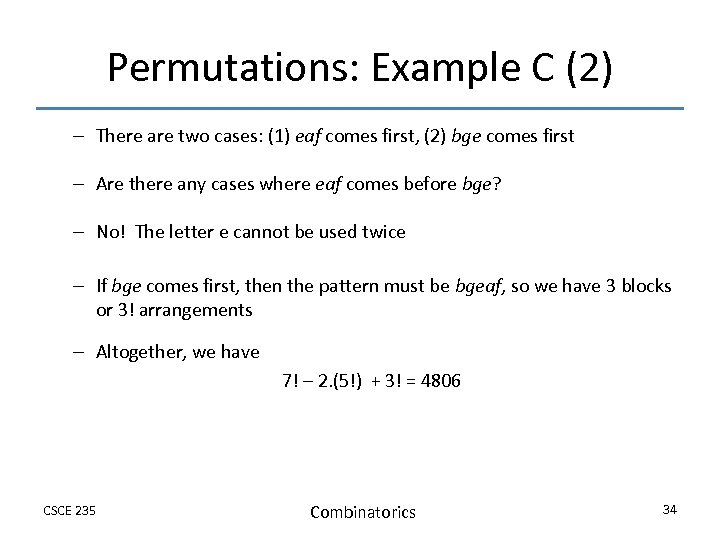Permutations: Example C (2) – There are two cases: (1) eaf comes first, (2) bge comes first – Are there any cases where eaf comes before bge? – No! The letter e cannot be used twice – If bge comes first, then the pattern must be bgeaf, so we have 3 blocks or 3! arrangements – Altogether, we have 7! – 2. (5!) + 3! = 4806 CSCE 235 Combinatorics 34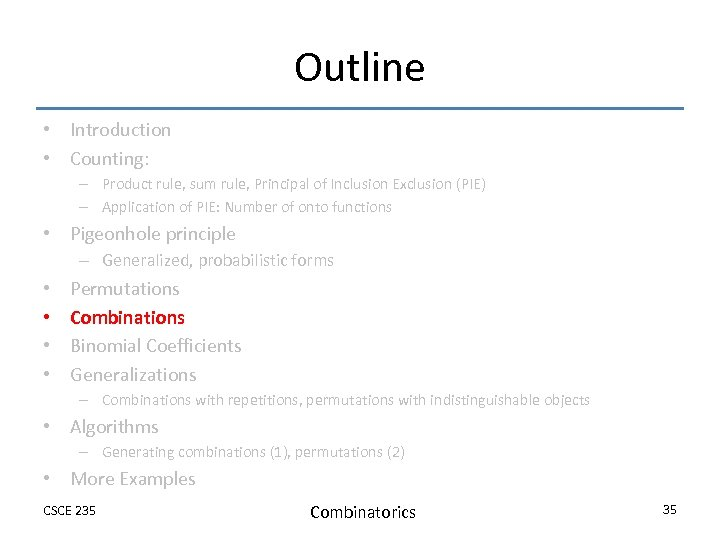Outline • Introduction • Counting: – Product rule, sum rule, Principal of Inclusion Exclusion (PIE) – Application of PIE: Number of onto functions • Pigeonhole principle – Generalized, probabilistic forms • • Permutations Combinations Binomial Coefficients Generalizations – Combinations with repetitions, permutations with indistinguishable objects • Algorithms – Generating combinations (1), permutations (2) • More Examples CSCE 235 Combinatorics 35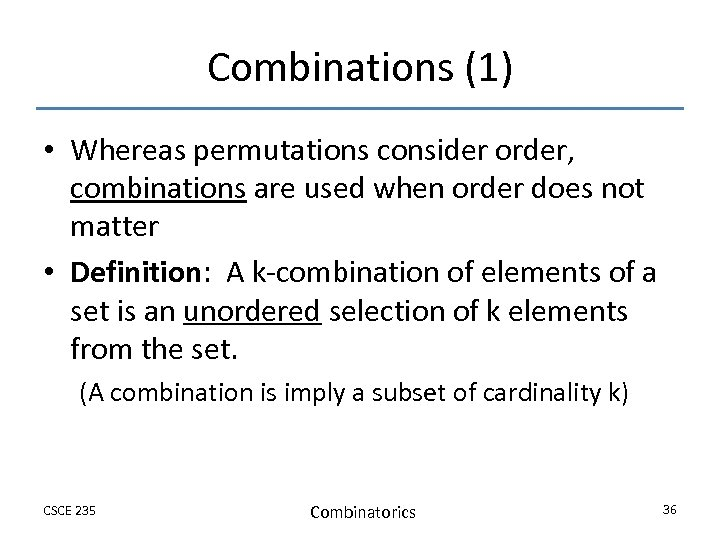Combinations (1) • Whereas permutations consider order, combinations are used when order does not matter • Definition: A k-combination of elements of a set is an unordered selection of k elements from the set. (A combination is imply a subset of cardinality k) CSCE 235 Combinatorics 36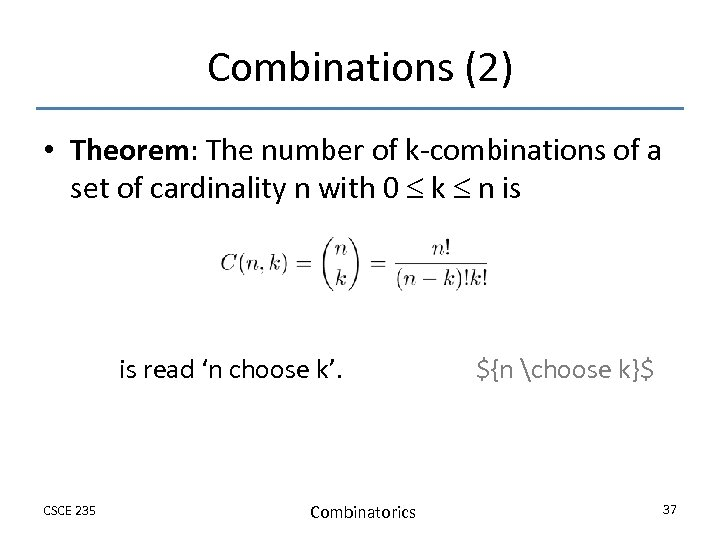Combinations (2) • Theorem: The number of k-combinations of a set of cardinality n with 0 k n is is read ‘n choose k’. CSCE 235 Combinatorics \${n choose k}\$ 37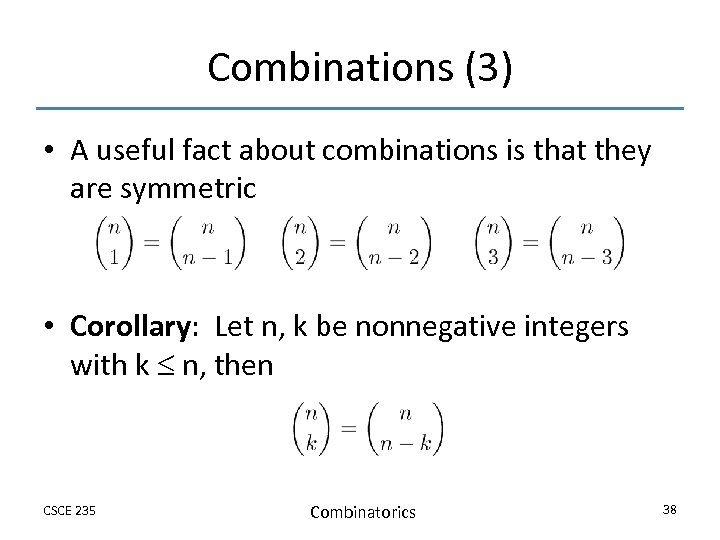Combinations (3) • A useful fact about combinations is that they are symmetric • Corollary: Let n, k be nonnegative integers with k n, then CSCE 235 Combinatorics 38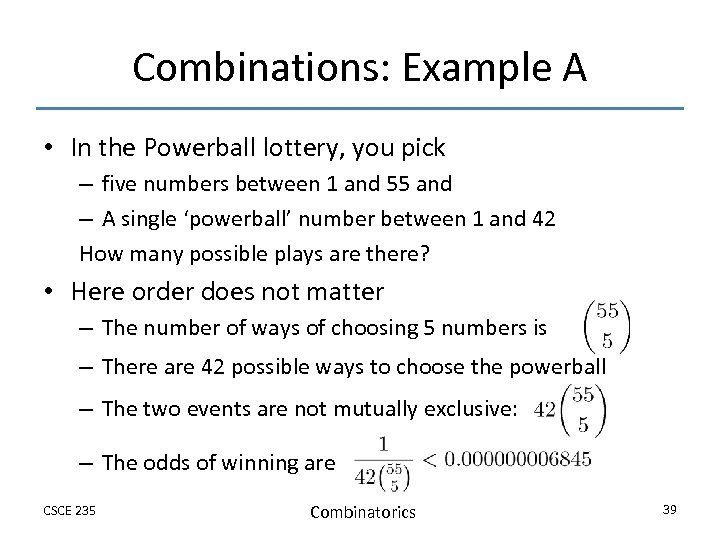Combinations: Example A • In the Powerball lottery, you pick – five numbers between 1 and 55 and – A single ‘powerball’ number between 1 and 42 How many possible plays are there? • Here order does not matter – The number of ways of choosing 5 numbers is – There are 42 possible ways to choose the powerball – The two events are not mutually exclusive: – The odds of winning are CSCE 235 Combinatorics 39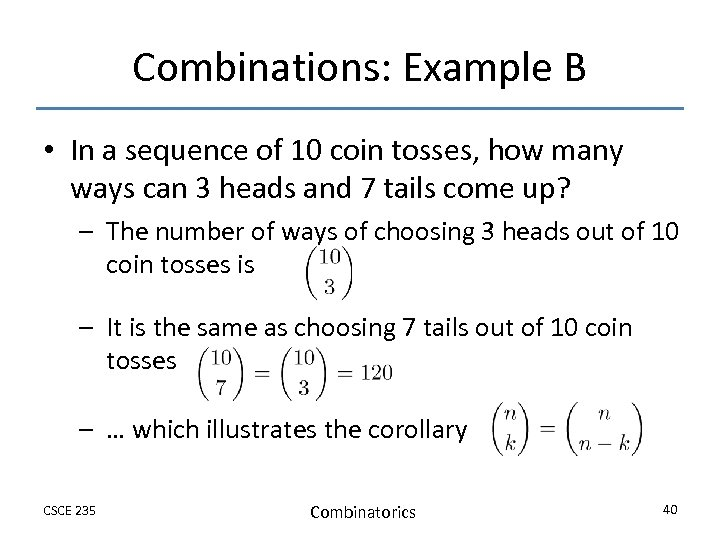Combinations: Example B • In a sequence of 10 coin tosses, how many ways can 3 heads and 7 tails come up? – The number of ways of choosing 3 heads out of 10 coin tosses is – It is the same as choosing 7 tails out of 10 coin tosses – … which illustrates the corollary CSCE 235 Combinatorics 40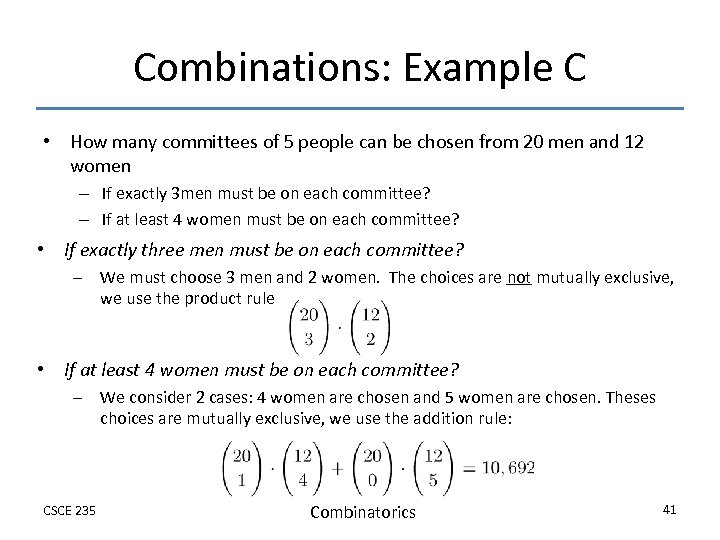Combinations: Example C • How many committees of 5 people can be chosen from 20 men and 12 women – If exactly 3 men must be on each committee? – If at least 4 women must be on each committee? • If exactly three men must be on each committee? – We must choose 3 men and 2 women. The choices are not mutually exclusive, we use the product rule • If at least 4 women must be on each committee? – We consider 2 cases: 4 women are chosen and 5 women are chosen. Theses choices are mutually exclusive, we use the addition rule: CSCE 235 Combinatorics 41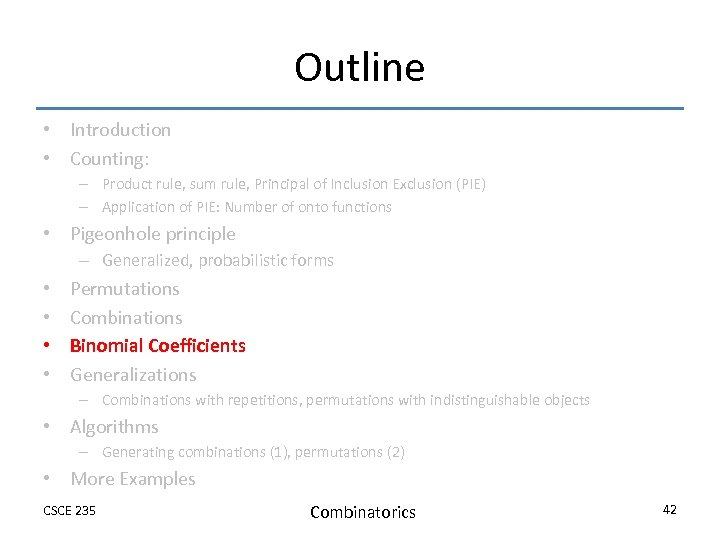Outline • Introduction • Counting: – Product rule, sum rule, Principal of Inclusion Exclusion (PIE) – Application of PIE: Number of onto functions • Pigeonhole principle – Generalized, probabilistic forms • • Permutations Combinations Binomial Coefficients Generalizations – Combinations with repetitions, permutations with indistinguishable objects • Algorithms – Generating combinations (1), permutations (2) • More Examples CSCE 235 Combinatorics 42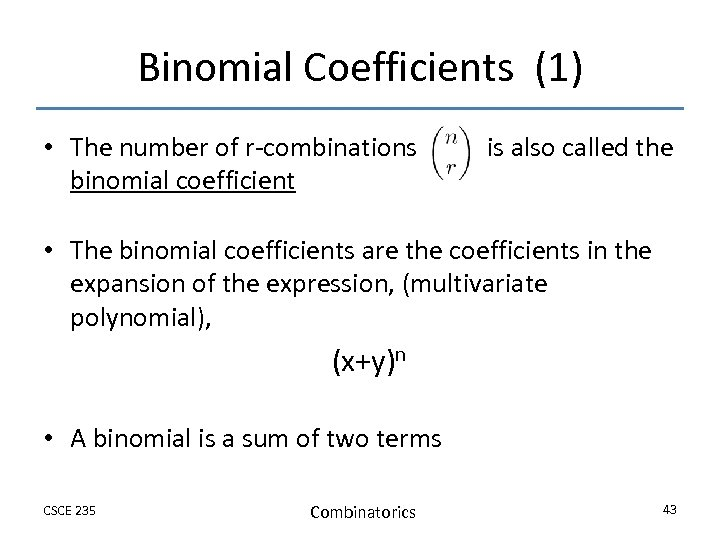Binomial Coefficients (1) • The number of r-combinations binomial coefficient is also called the • The binomial coefficients are the coefficients in the expansion of the expression, (multivariate polynomial), (x+y)n • A binomial is a sum of two terms CSCE 235 Combinatorics 43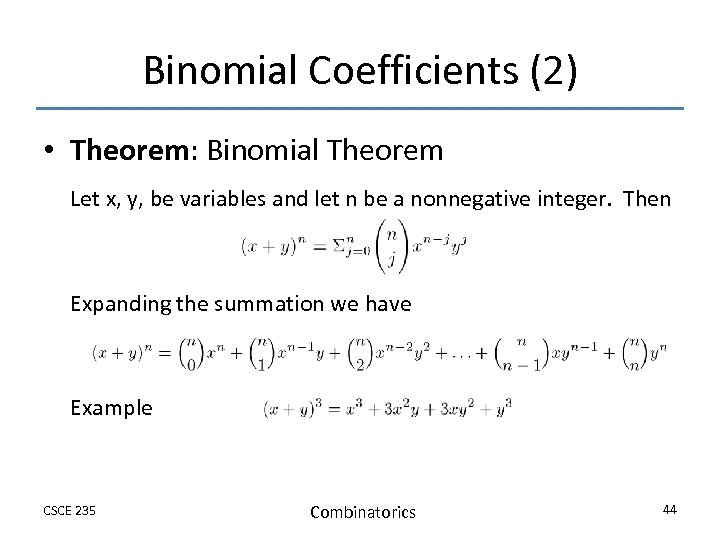Binomial Coefficients (2) • Theorem: Binomial Theorem Let x, y, be variables and let n be a nonnegative integer. Then Expanding the summation we have Example CSCE 235 Combinatorics 44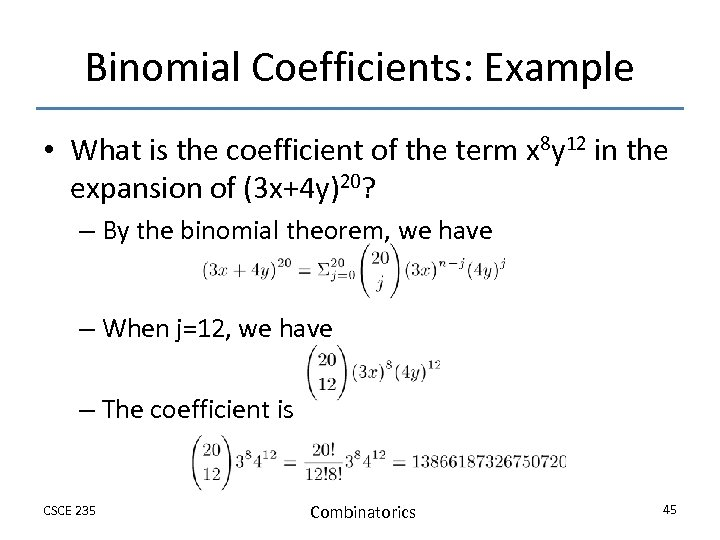Binomial Coefficients: Example • What is the coefficient of the term x 8 y 12 in the expansion of (3 x+4 y)20? – By the binomial theorem, we have – When j=12, we have – The coefficient is CSCE 235 Combinatorics 45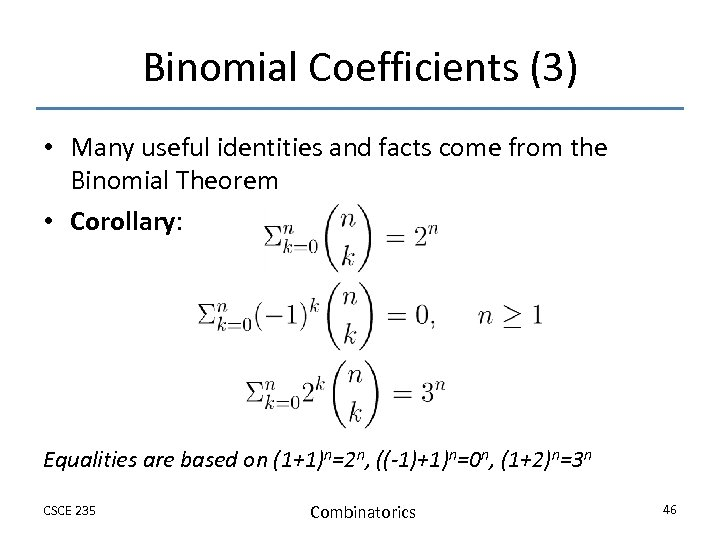Binomial Coefficients (3) • Many useful identities and facts come from the Binomial Theorem • Corollary: Equalities are based on (1+1)n=2 n, ((-1)+1)n=0 n, (1+2)n=3 n CSCE 235 Combinatorics 46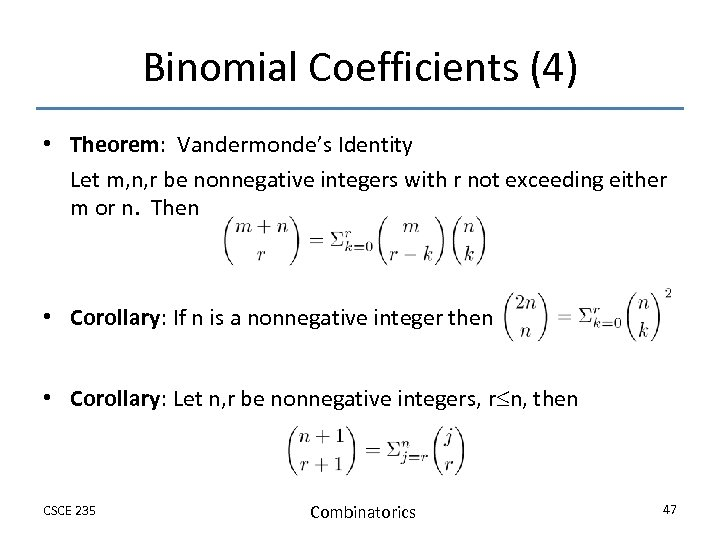Binomial Coefficients (4) • Theorem: Vandermonde’s Identity Let m, n, r be nonnegative integers with r not exceeding either m or n. Then • Corollary: If n is a nonnegative integer then • Corollary: Let n, r be nonnegative integers, r n, then CSCE 235 Combinatorics 47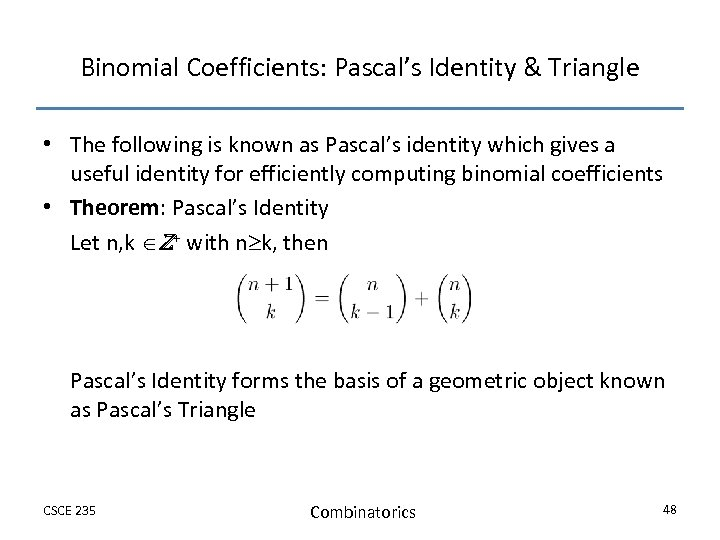Binomial Coefficients: Pascal’s Identity & Triangle • The following is known as Pascal’s identity which gives a useful identity for efficiently computing binomial coefficients • Theorem: Pascal’s Identity Let n, k Z+ with n k, then Pascal’s Identity forms the basis of a geometric object known as Pascal’s Triangle CSCE 235 Combinatorics 48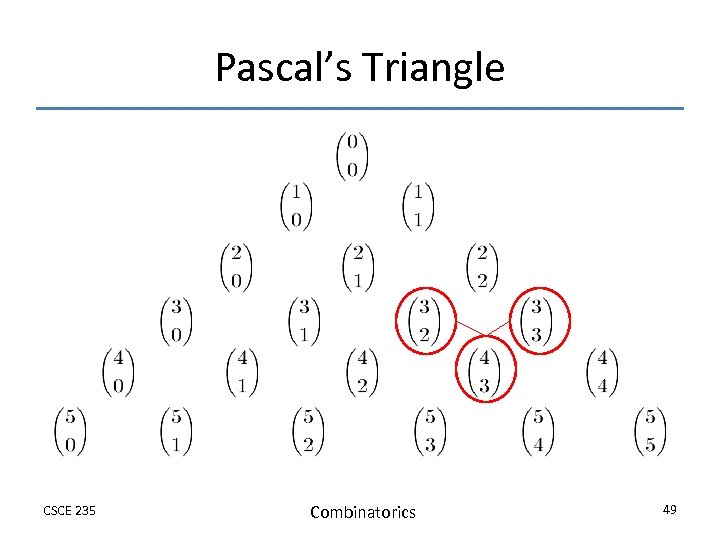Pascal’s Triangle CSCE 235 Combinatorics 49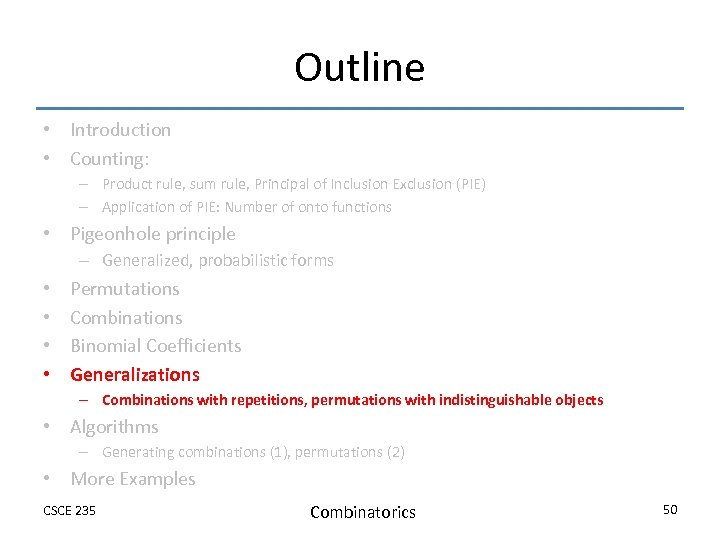Outline • Introduction • Counting: – Product rule, sum rule, Principal of Inclusion Exclusion (PIE) – Application of PIE: Number of onto functions • Pigeonhole principle – Generalized, probabilistic forms • • Permutations Combinations Binomial Coefficients Generalizations – Combinations with repetitions, permutations with indistinguishable objects • Algorithms – Generating combinations (1), permutations (2) • More Examples CSCE 235 Combinatorics 50Generalized Combinations & Permutations (1) • Sometimes, we are interested in permutations and combinations in which repetitions are allowed • Theorem: The number of r-permutations of a set of n objects with repetition allowed is nr …which is easily obtained by the product rule • Theorem: There are r-combinations from a set with n elements when repetition of elements is allowed CSCE 235 Combinatorics 51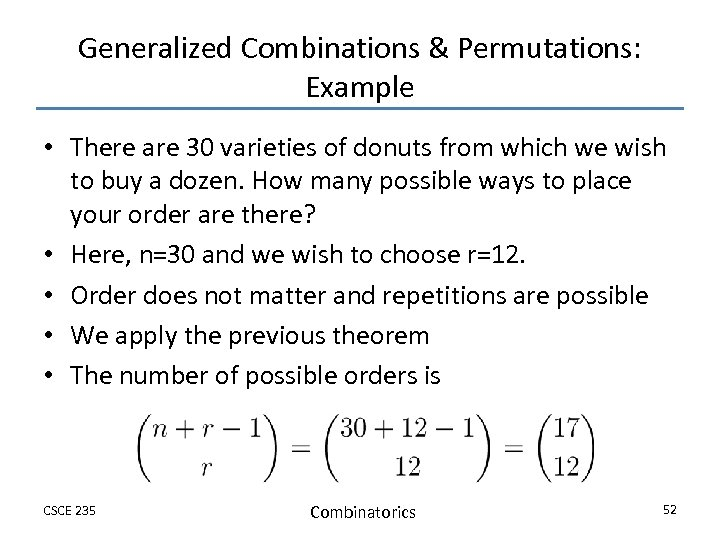Generalized Combinations & Permutations: Example • There are 30 varieties of donuts from which we wish to buy a dozen. How many possible ways to place your order are there? • Here, n=30 and we wish to choose r=12. • Order does not matter and repetitions are possible • We apply the previous theorem • The number of possible orders is CSCE 235 Combinatorics 52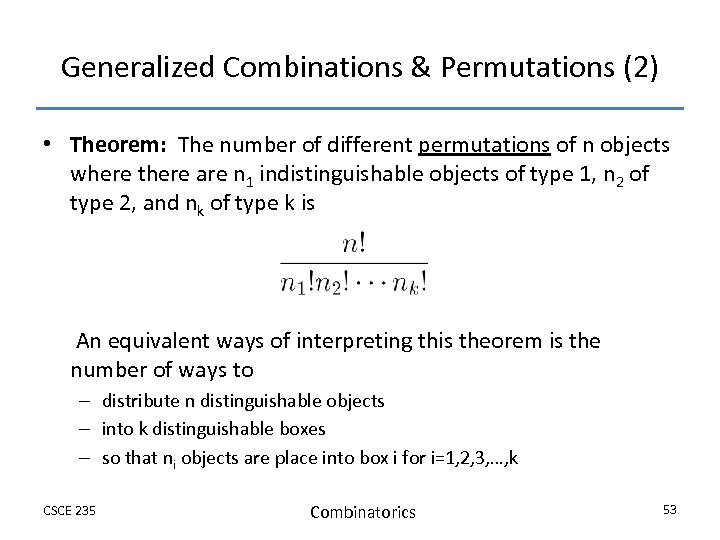Generalized Combinations & Permutations (2) • Theorem: The number of different permutations of n objects where there are n 1 indistinguishable objects of type 1, n 2 of type 2, and nk of type k is An equivalent ways of interpreting this theorem is the number of ways to – distribute n distinguishable objects – into k distinguishable boxes – so that ni objects are place into box i for i=1, 2, 3, …, k CSCE 235 Combinatorics 53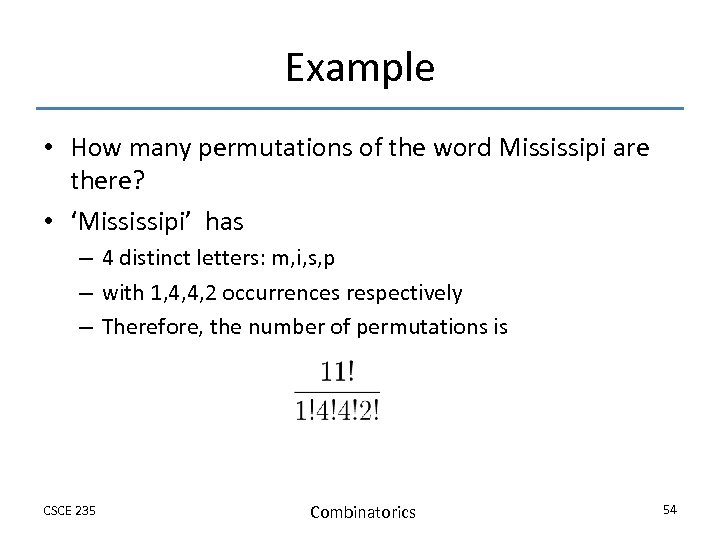Example • How many permutations of the word Mississipi are there? • ‘Mississipi’ has – 4 distinct letters: m, i, s, p – with 1, 4, 4, 2 occurrences respectively – Therefore, the number of permutations is CSCE 235 Combinatorics 54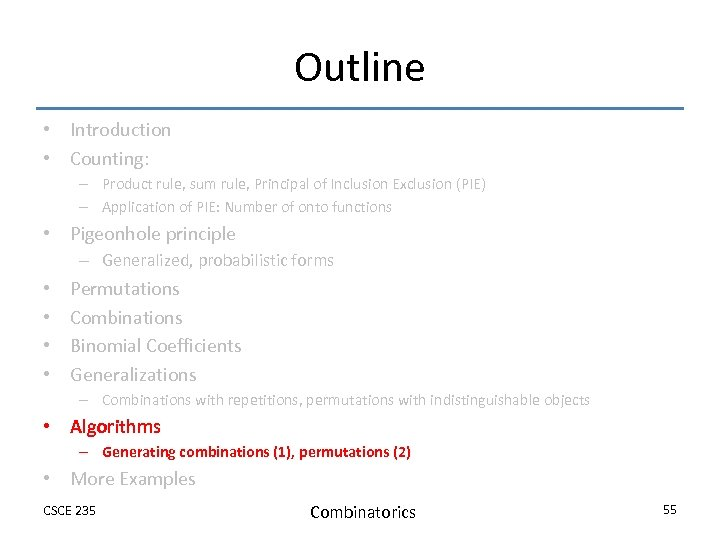Outline • Introduction • Counting: – Product rule, sum rule, Principal of Inclusion Exclusion (PIE) – Application of PIE: Number of onto functions • Pigeonhole principle – Generalized, probabilistic forms • • Permutations Combinations Binomial Coefficients Generalizations – Combinations with repetitions, permutations with indistinguishable objects • Algorithms – Generating combinations (1), permutations (2) • More Examples CSCE 235 Combinatorics 55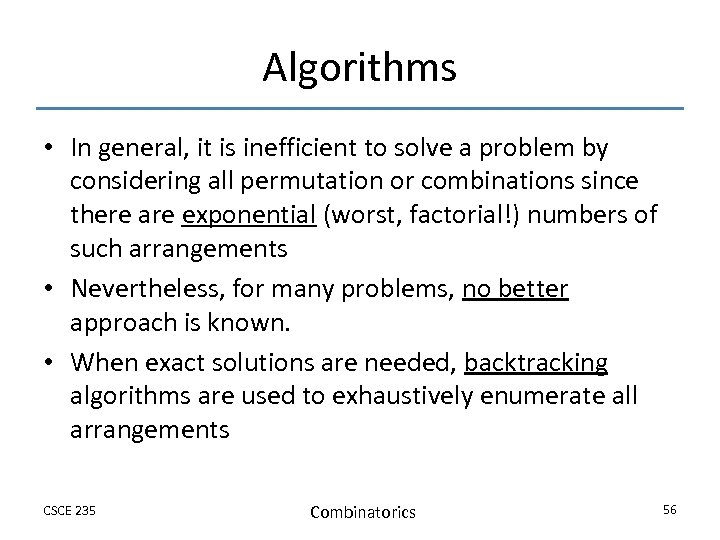Algorithms • In general, it is inefficient to solve a problem by considering all permutation or combinations since there are exponential (worst, factorial!) numbers of such arrangements • Nevertheless, for many problems, no better approach is known. • When exact solutions are needed, backtracking algorithms are used to exhaustively enumerate all arrangements CSCE 235 Combinatorics 56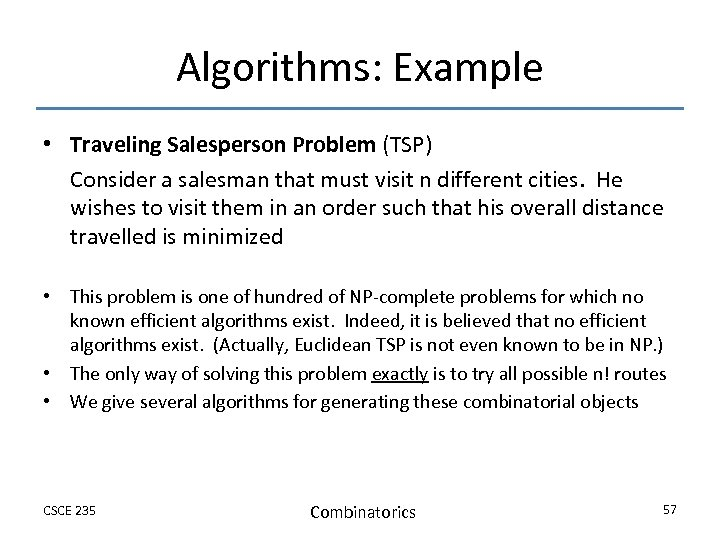Algorithms: Example • Traveling Salesperson Problem (TSP) Consider a salesman that must visit n different cities. He wishes to visit them in an order such that his overall distance travelled is minimized • This problem is one of hundred of NP-complete problems for which no known efficient algorithms exist. Indeed, it is believed that no efficient algorithms exist. (Actually, Euclidean TSP is not even known to be in NP. ) • The only way of solving this problem exactly is to try all possible n! routes • We give several algorithms for generating these combinatorial objects CSCE 235 Combinatorics 57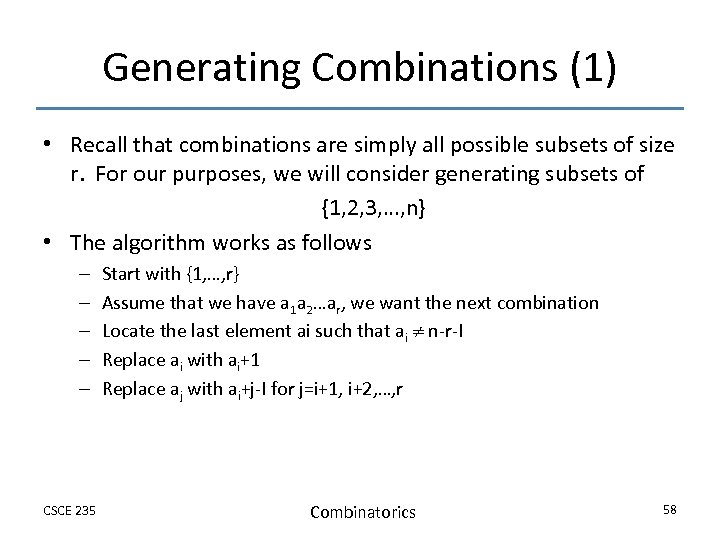Generating Combinations (1) • Recall that combinations are simply all possible subsets of size r. For our purposes, we will consider generating subsets of {1, 2, 3, …, n} • The algorithm works as follows – – – CSCE 235 Start with {1, …, r} Assume that we have a 1 a 2…ar, we want the next combination Locate the last element ai such that ai n-r-I Replace ai with ai+1 Replace aj with ai+j-I for j=i+1, i+2, …, r Combinatorics 58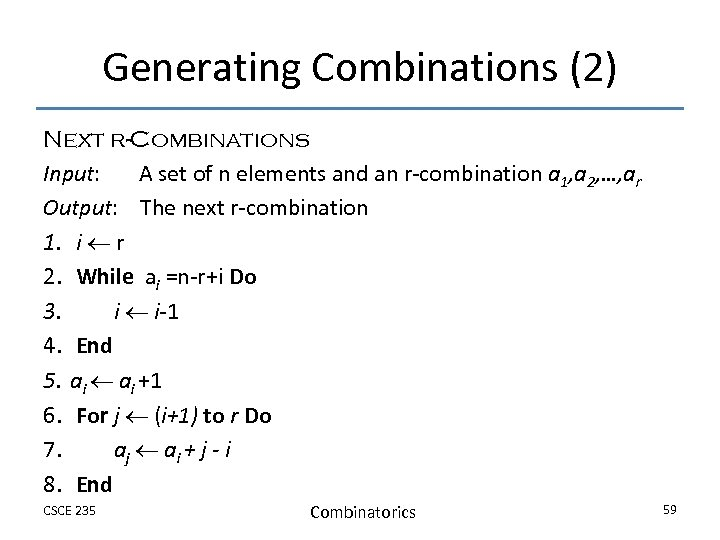Generating Combinations (2) Next r-Combinations Input: A set of n elements and an r-combination a 1, a 2, …, ar Output: The next r-combination 1. i r 2. While ai =n-r+i Do 3. i i-1 4. End 5. ai +1 6. For j (i+1) to r Do 7. aj ai + j - i 8. End CSCE 235 Combinatorics 59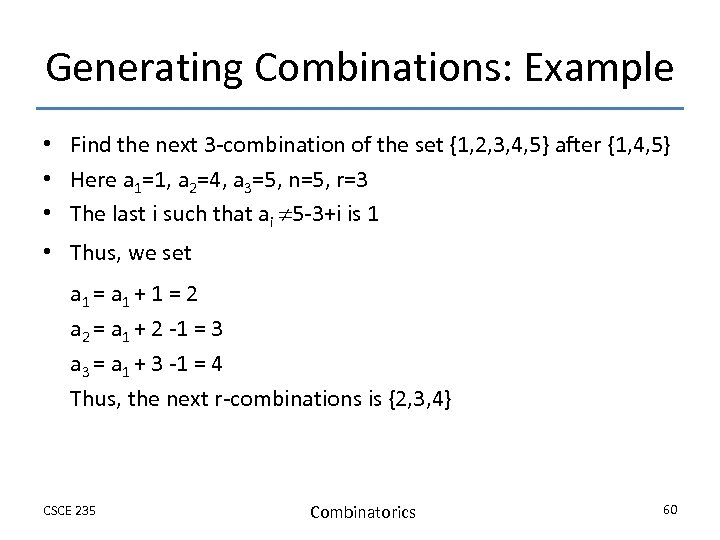Generating Combinations: Example • Find the next 3 -combination of the set {1, 2, 3, 4, 5} after {1, 4, 5} • Here a 1=1, a 2=4, a 3=5, n=5, r=3 • The last i such that ai 5 -3+i is 1 • Thus, we set a 1 = a 1 + 1 = 2 a 2 = a 1 + 2 -1 = 3 a 3 = a 1 + 3 -1 = 4 Thus, the next r-combinations is {2, 3, 4} CSCE 235 Combinatorics 60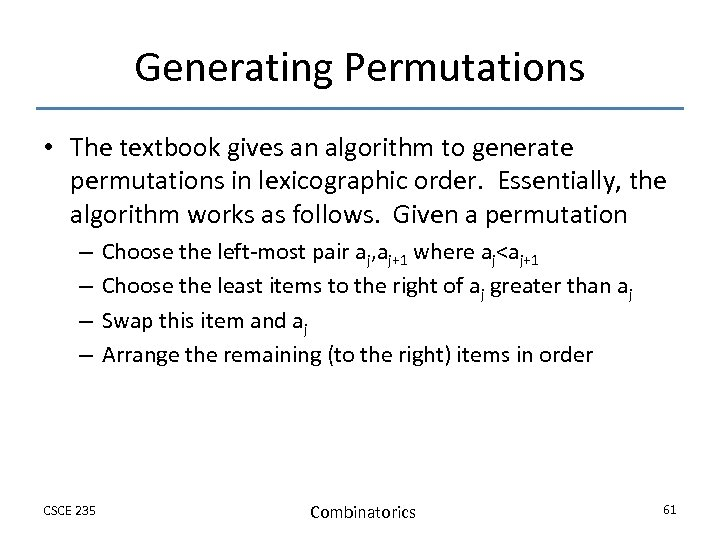Generating Permutations • The textbook gives an algorithm to generate permutations in lexicographic order. Essentially, the algorithm works as follows. Given a permutation – – CSCE 235 Choose the left-most pair aj, aj+1 where aj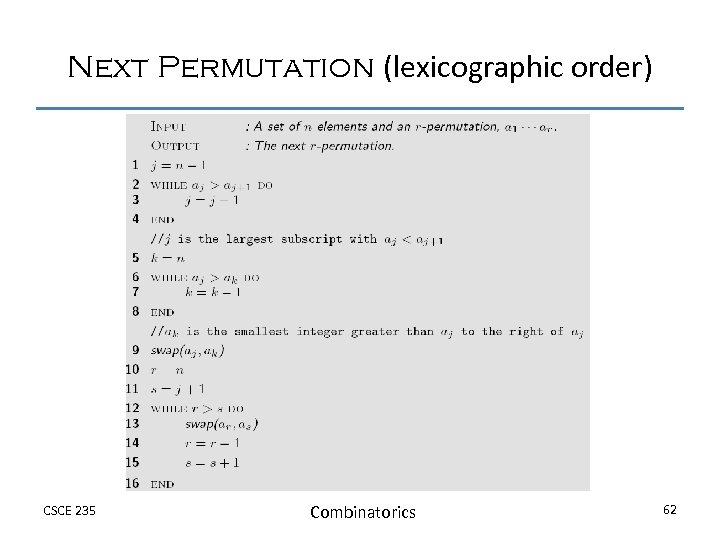Next Permutation (lexicographic order) CSCE 235 Combinatorics 62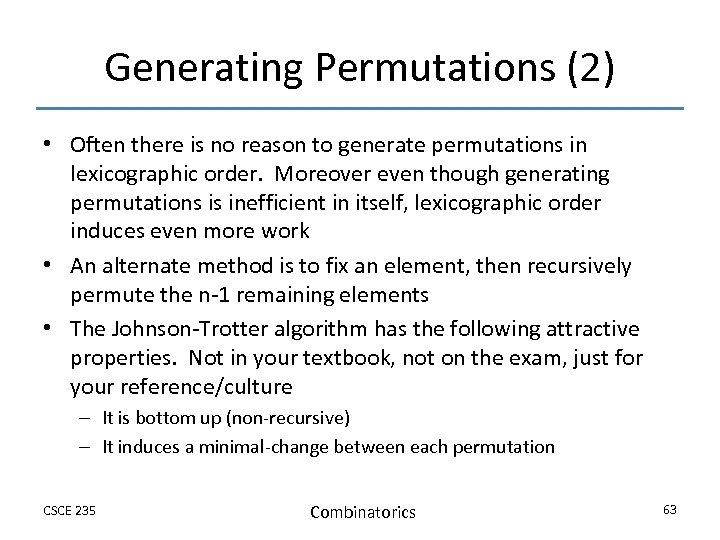Generating Permutations (2) • Often there is no reason to generate permutations in lexicographic order. Moreover even though generating permutations is inefficient in itself, lexicographic order induces even more work • An alternate method is to fix an element, then recursively permute the n-1 remaining elements • The Johnson-Trotter algorithm has the following attractive properties. Not in your textbook, not on the exam, just for your reference/culture – It is bottom up (non-recursive) – It induces a minimal-change between each permutation CSCE 235 Combinatorics 63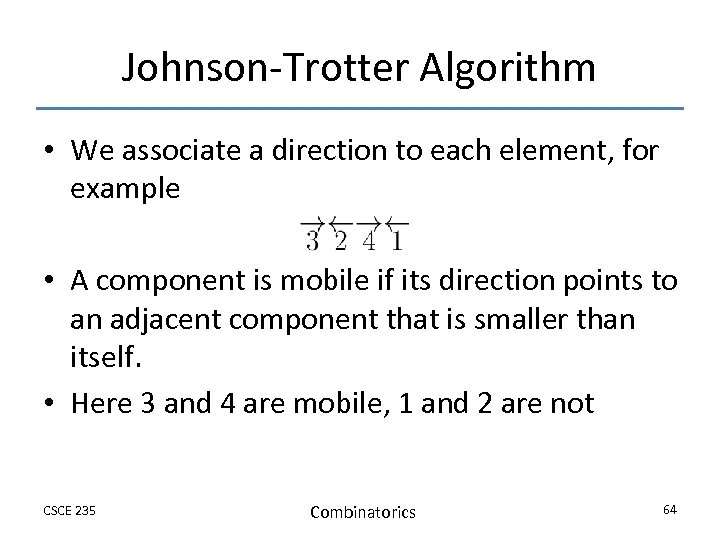Johnson-Trotter Algorithm • We associate a direction to each element, for example • A component is mobile if its direction points to an adjacent component that is smaller than itself. • Here 3 and 4 are mobile, 1 and 2 are not CSCE 235 Combinatorics 64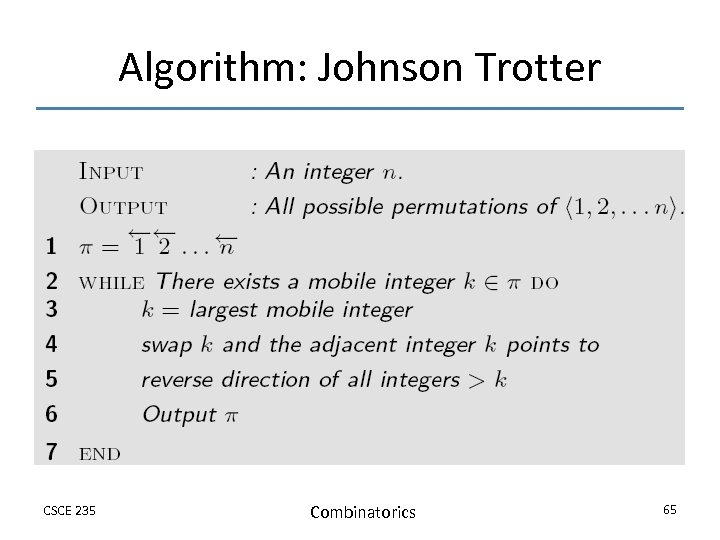Algorithm: Johnson Trotter CSCE 235 Combinatorics 65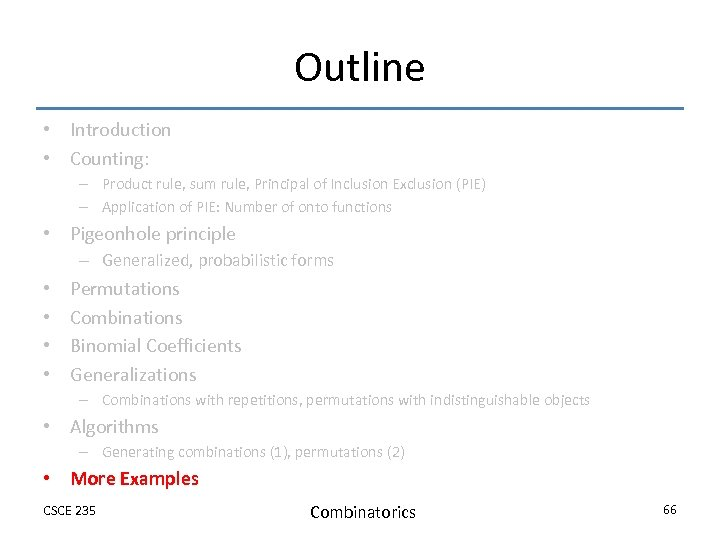Outline • Introduction • Counting: – Product rule, sum rule, Principal of Inclusion Exclusion (PIE) – Application of PIE: Number of onto functions • Pigeonhole principle – Generalized, probabilistic forms • • Permutations Combinations Binomial Coefficients Generalizations – Combinations with repetitions, permutations with indistinguishable objects • Algorithms – Generating combinations (1), permutations (2) • More Examples CSCE 235 Combinatorics 66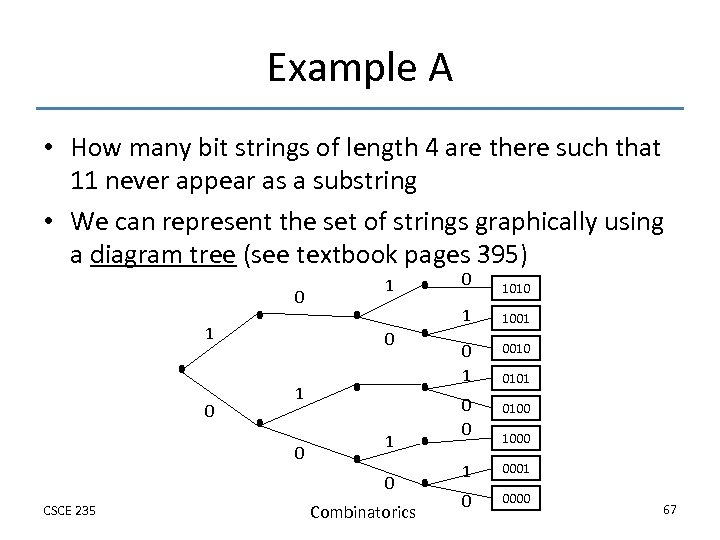Example A • How many bit strings of length 4 are there such that 11 never appear as a substring • We can represent the set of strings graphically using a diagram tree (see textbook pages 395) 0 1 0 1 0 CSCE 235 Combinatorics 0 1010 1 1 1001 0 1 0010 0 0 0100 1 0001 0 0000 0101 1000 67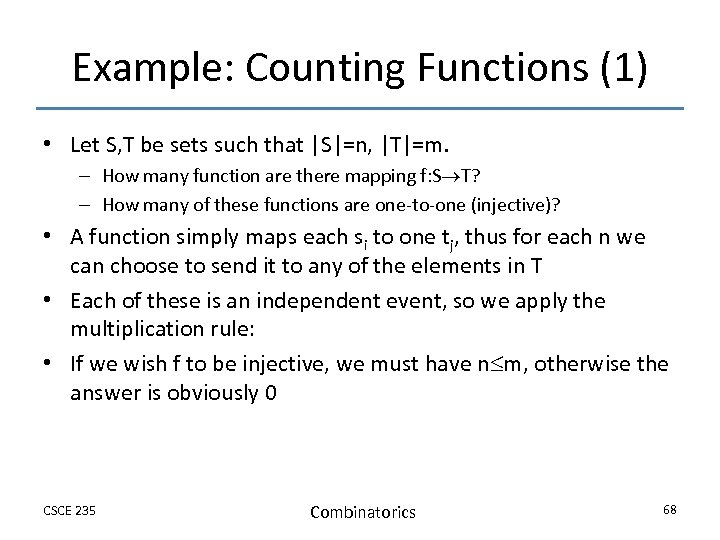Example: Counting Functions (1) • Let S, T be sets such that |S|=n, |T|=m. – How many function are there mapping f: S T? – How many of these functions are one-to-one (injective)? • A function simply maps each si to one tj, thus for each n we can choose to send it to any of the elements in T • Each of these is an independent event, so we apply the multiplication rule: • If we wish f to be injective, we must have n m, otherwise the answer is obviously 0 CSCE 235 Combinatorics 68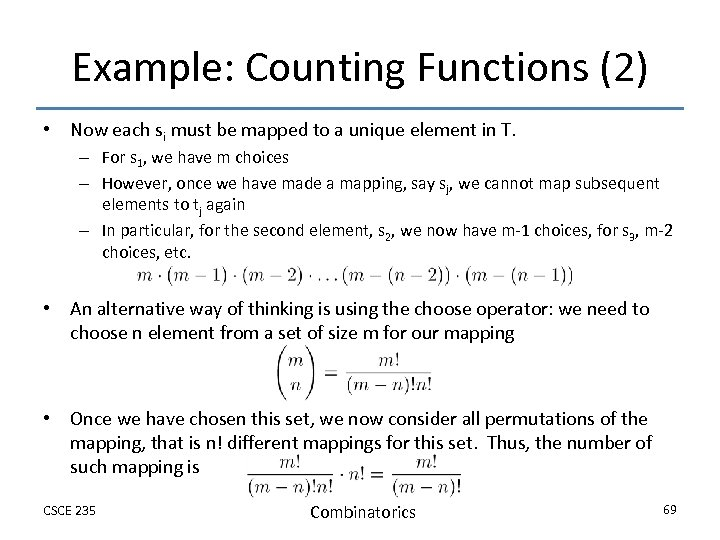Example: Counting Functions (2) • Now each si must be mapped to a unique element in T. – For s 1, we have m choices – However, once we have made a mapping, say sj, we cannot map subsequent elements to tj again – In particular, for the second element, s 2, we now have m-1 choices, for s 3, m-2 choices, etc. • An alternative way of thinking is using the choose operator: we need to choose n element from a set of size m for our mapping • Once we have chosen this set, we now consider all permutations of the mapping, that is n! different mappings for this set. Thus, the number of such mapping is CSCE 235 Combinatorics 69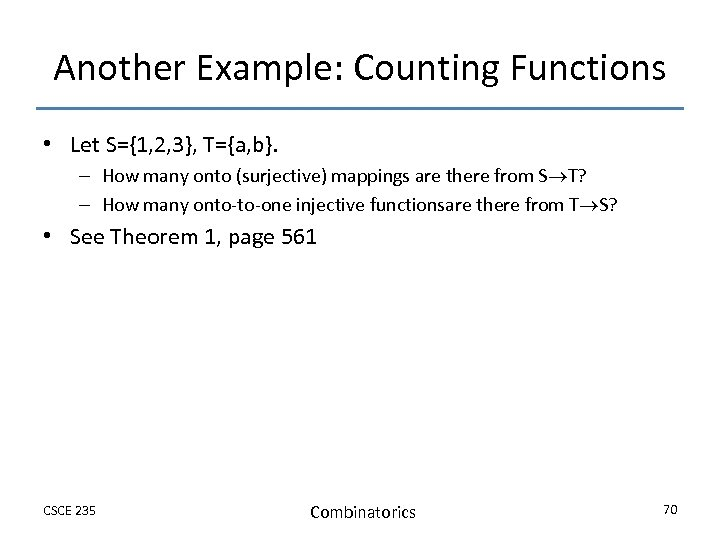Another Example: Counting Functions • Let S={1, 2, 3}, T={a, b}. – How many onto (surjective) mappings are there from S T? – How many onto-to-one injective functionsare there from T S? • See Theorem 1, page 561 CSCE 235 Combinatorics 70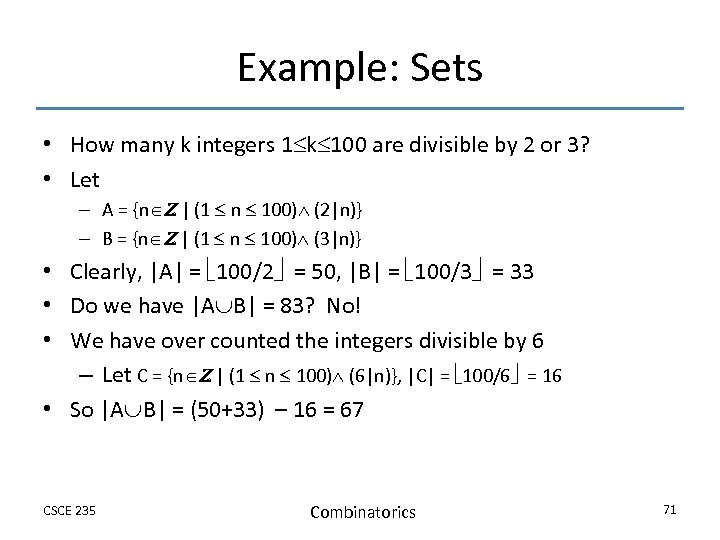Example: Sets • How many k integers 1 k 100 are divisible by 2 or 3? • Let – A = {n Z | (1 n 100) (2|n)} – B = {n Z | (1 n 100) (3|n)} • Clearly, |A| = 100/2 = 50, |B| = 100/3 = 33 • Do we have |A B| = 83? No! • We have over counted the integers divisible by 6 – Let C = {n Z | (1 n 100) (6|n)}, |C| = 100/6 = 16 • So |A B| = (50+33) – 16 = 67 CSCE 235 Combinatorics 71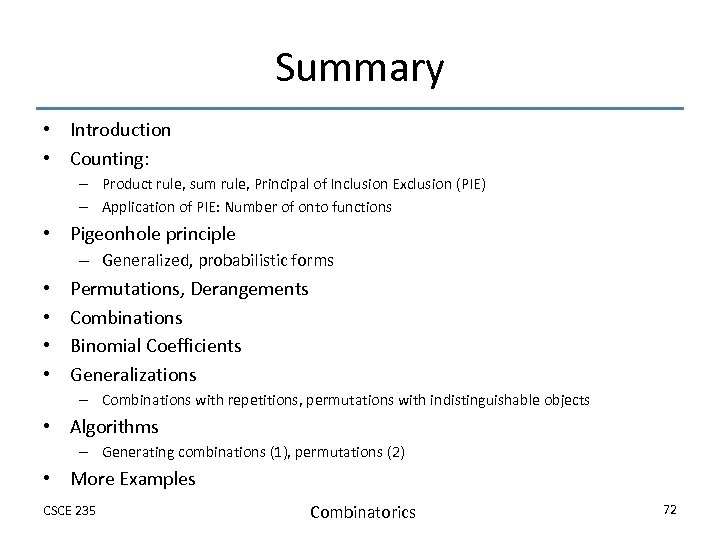Summary • Introduction • Counting: – Product rule, sum rule, Principal of Inclusion Exclusion (PIE) – Application of PIE: Number of onto functions • Pigeonhole principle – Generalized, probabilistic forms • • Permutations, Derangements Combinations Binomial Coefficients Generalizations – Combinations with repetitions, permutations with indistinguishable objects • Algorithms – Generating combinations (1), permutations (2) • More Examples CSCE 235 Combinatorics 72